• MTI动目标指示和MTD动目标检测

千次阅读 多人点赞 2021-04-14 23:56:37
MTD（moving targets detection）雷达系统中区分运动目标和杂波在于它们...动目标检测(MTD)不仅能滤除杂波，而且还可以将不同运动速度的目标区分开来，从而大大改善了在杂波背景下检测运动目标的能力。 后续补充 ...

MTD （moving targets detection）是带通滤波器组，也就是多个输入多个输出，可以用FIR 组实现，但是一般用FFT 实现，即对不同脉冲组回波信号的相同距离单元做FFT 处理，N 个输入得到N 个输出，CACFAR 既是对这N 个输出进行检测和判断目标！若存在目标，这N 个输出中比出现一个峰值最大的数，则这个可能就是目标的位置信息，根据位置信息，即可以得到运动目标的多普勒值。MTD雷达系统中区分运动目标和杂波在于它们速度上的差别。由于速度不同而引起回波信号的多普勒频率不相等，所以可以通过多普勒频率的不同将杂波和运动目标区分开来。动目标检测(MTD)不仅能滤除杂波，而且还可以将不同运动速度的目标区分开来，从而大大改善了在杂波背景下检测运动目标的能力。

MATLAB仿真：

1，雷达信号做脉冲压缩

% ===================================================================================%
%                                    雷达参数                                       %
% ===================================================================================%
C=3.0e8;  %光速(m/s)
RF=3.140e9/2;  %雷达射频 1.57GHz
Lambda=C/RF;%雷达工作波长
PulseNumber=16;   %回波脉冲数
BandWidth=2.0e6;  %发射信号带宽 带宽B=1/τ，τ是脉冲宽度
TimeWidth=42.0e-6; %发射信号时宽
PRT=240e-6;   % 雷达发射脉冲重复周期(s),240us对应1/2*240*300=36000米最大无模糊距离
PRF=1/PRT;
Fs=2.0e6;  %采样频率
NoisePower=-12;%(dB);%噪声功率（目标为0dB）
% ---------------------------------------------------------------%
SampleNumber=fix(Fs*PRT);%计算一个脉冲周期的采样点数480；
TotalNumber=SampleNumber*PulseNumber;%总的采样点数480*16=；
BlindNumber=fix(Fs*TimeWidth);%计算一个脉冲周期的盲区-遮挡样点数；
%===================================================================================%
%                                    目标参数                                       %
%===================================================================================%
TargetNumber=4;%目标个数
SigPower(1:TargetNumber)=[1 1 1 0.25];%目标功率,无量纲
TargetDistance(1:TargetNumber)=[3000 8025 15800 8025];%目标距离,单位m   距离参数为[3000 8025 9000+(Y*10+Z)*200 8025]
DelayNumber(1:TargetNumber)=fix(Fs*2*TargetDistance(1:TargetNumber)/C);% 把目标距离换算成采样点（距离门） fix函数向0靠拢取整
TargetVelocity(1:TargetNumber)=[50 0 204 100];%目标径向速度 单位m/s   速度参数为[50 0 (Y*10+X+Z)*6 100]
TargetFd(1:TargetNumber)=2*TargetVelocity(1:TargetNumber)/Lambda; %计算目标多卜勒频移2v/λ

%====================================================================================%
%                                   产生线性调频信号                                  %
%====================================================================================%
number=fix(Fs*TimeWidth);%回波的采样点数=脉压系数长度=暂态点数目+1
if rem(number,2)~=0  %rem求余
number=number+1;
end   %把number变为偶数

for i=-fix(number/2):fix(number/2)-1
Chirp(i+fix(number/2)+1)=exp(j*(pi*(BandWidth/TimeWidth)*(i/Fs)^2));%exp(j*fi)*，产生复数矩阵Chirp
end
coeff=conj(fliplr(Chirp));%把Chirp矩阵翻转并把复数共轭，产生脉压系数
figure(1);%脉压系数的实部
plot(real(Chirp));axis([0 90 -1.5 1.5]);title('脉压系数实部');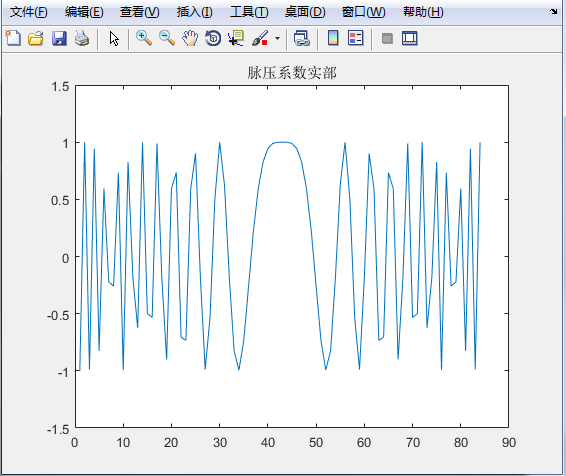2，生成目标信号回波

%-------------------------产生目标回波串-----------------------------------------------------------------------------------------%
%-------------------------产生前3个目标的回波串-------%
SignalAll=zeros(1,TotalNumber);%所有脉冲的信号,先填0
for k=1:TargetNumber-1 % 依次产生各个目标
SignalTemp=zeros(1,SampleNumber);% 一个PRT
SignalTemp(DelayNumber(k)+1:DelayNumber(k)+number)=sqrt(SigPower(k))*Chirp;%一个脉冲的1个目标（未加多普勒速度）(DelayNumber(k)+1):(DelayNumber(k)+number)
Signal=zeros(1,TotalNumber);
for i=1:PulseNumber % 16个回波脉冲
Signal((i-1)*SampleNumber+1:i*SampleNumber)=SignalTemp; %每个目标把16个SignalTemp排在一起
end
FreqMove=exp(j*2*pi*TargetFd(k)*(0:TotalNumber-1)/Fs);%目标的多普勒速度*时间=目标的多普勒相移
Signal=Signal.*FreqMove;%加上多普勒速度后的16个脉冲1个目标
SignalAll=SignalAll+Signal;%加上多普勒速度后的16个脉冲4个目标
end
% %-------------------------产生第4个目标的回波串-------%
fi=pi/3;
SignalTemp=zeros(1,SampleNumber);% 一个脉冲
SignalTemp(DelayNumber(4)+1:DelayNumber(4)+number)=sqrt(SigPower(4))*exp(j*fi)*Chirp;%一个脉冲的1个目标（未加多普勒速度）
Signal=zeros(1,TotalNumber);
for i=1:PulseNumber
Signal((i-1)*SampleNumber+1:i*SampleNumber)=SignalTemp;
end
FreqMove=exp(j*2*pi*TargetFd(4)*(0:TotalNumber-1)/Fs);%目标的多普勒速度*时间=目标的多普勒相移
Signal=Signal.*FreqMove;
SignalAll=SignalAll+Signal;

figure(2);
subplot(2,1,1);plot(real(SignalAll),'r-');title('目标信号的实部');grid on;zoom on;
subplot(2,1,2);plot(imag(SignalAll));title('目标信号的虚部');grid on;zoom on;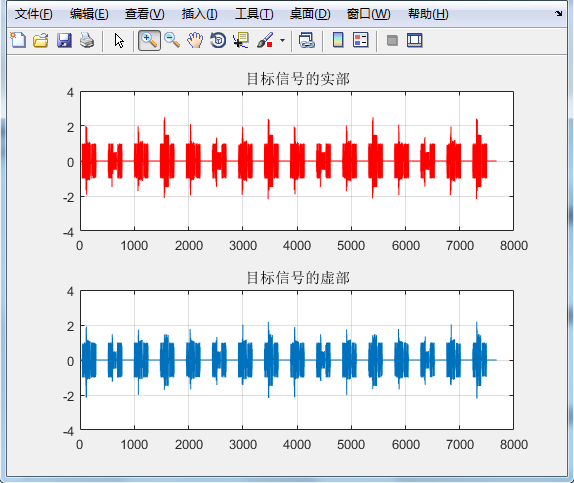3，产生系统噪声信号

%====================================================================================%
%                                   产生系统噪声信号                                  %
%====================================================================================%
SystemNoise=normrnd(0,10^(NoisePower/10),1,TotalNumber)+j*normrnd(0,10^(NoisePower/10),1,TotalNumber);
%均值为0，标准差为10^(NoisePower/10)的噪声
%====================================================================================%
%                                   总的回波信号                                     %
%====================================================================================%
Echo=SignalAll+SystemNoise;% +SeaClutter+TerraClutter，加噪声之后的回波
for i=1:PulseNumber   %在接收机闭锁期,接收的回波为0
Echo((i-1)*SampleNumber+1:(i-1)*SampleNumber+number)=0; %发射时接收为0
end
figure(3);%加噪声之后的总回波信号
subplot(2,1,1);plot(real(Echo),'r-');title('总回波信号的实部,闭锁期为0');
subplot(2,1,2);plot(imag(Echo));title('总回波信号的虚部,闭锁期为0');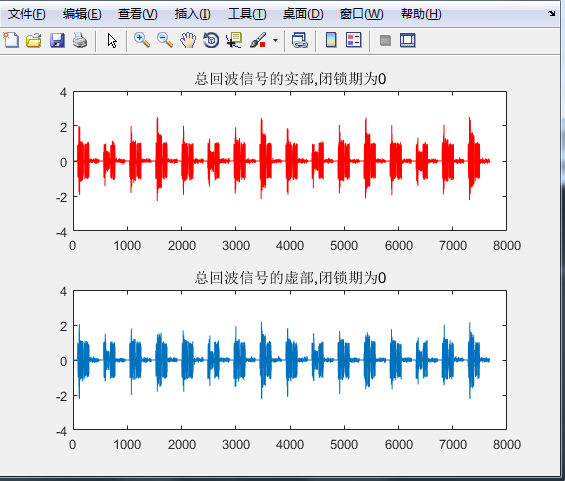4，时域脉冲压缩和频域脉冲压缩

%================================时域脉压=================================%
pc_time0=conv(Echo,coeff);%pc_time0为Echo和coeff的卷积
pc_time1=pc_time0(number:TotalNumber+number-1);%去掉暂态点 number-1个
figure(4);%时域脉压结果的幅度
subplot(2,1,1);plot(abs(pc_time0),'r-');title('时域脉压结果的幅度,有暂态点');%pc_time0的模的曲线
subplot(2,1,2);plot(abs(pc_time1));title('时域脉压结果的幅度,无暂态点');%pc_time1的模的曲线
% ================================频域脉压=================================%
Echo_fft=fft(Echo,8192);%理应进行TotalNumber+number-1点FFT,但为了提高运算速度,进行了8192点的FFT
coeff_fft=fft(coeff,8192);
pc_fft=Echo_fft.*coeff_fft;
pc_freq0=ifft(pc_fft);
figure(5);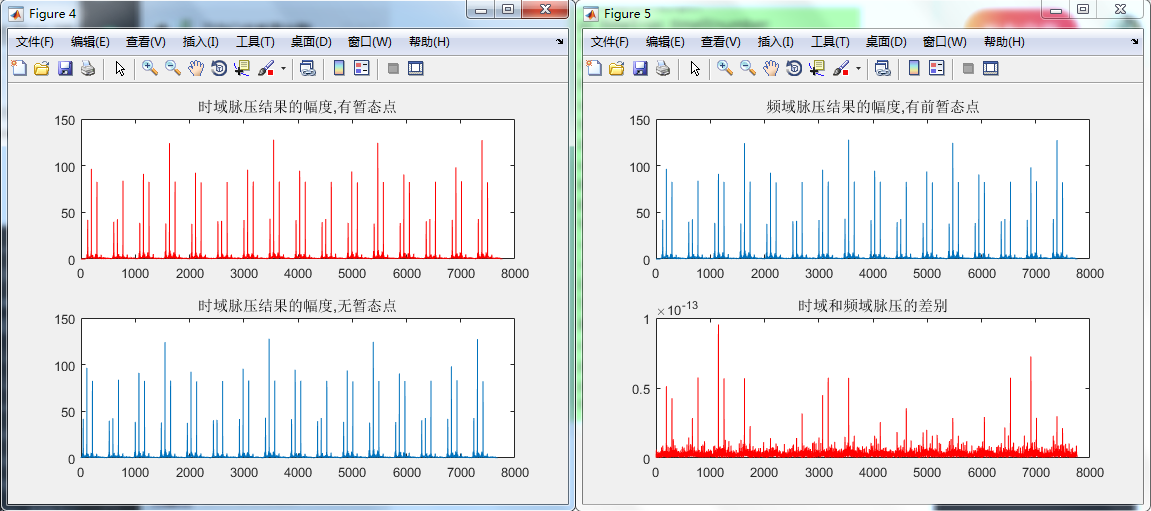按照脉冲号、距离门号重排数据

pc_freq1=pc_freq0(number:TotalNumber+number-1);%去掉暂态点 number-1个,后填充点若干(8192-number+1-TotalNumber)
% ================按照脉冲号、距离门号重排数据=================================%
for i=1:PulseNumber
pc(i,1:SampleNumber)=pc_freq1((i-1)*SampleNumber+1:i*SampleNumber);%每个PRT为一行，每行480个采样点的数据
end
figure(6);
plot(abs(pc(1,:)));title('频域脉压结果的幅度,没有暂态点');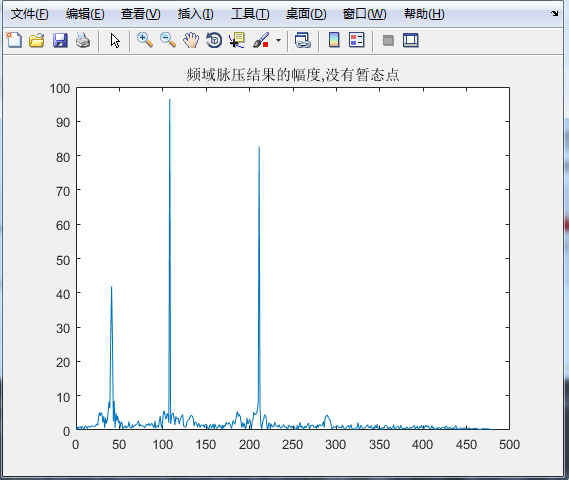5，MTI和MTD

% ================MTI（动目标显示）,对消静止目标和低速目标---可抑制杂波=================================%
for i=1:PulseNumber-1  %滑动对消，少了一个脉冲
mti(i,:)=pc(i+1,:)-pc(i,:);
end
figure(7);
mesh(abs(mti));title('MTI  result');

% ================MTD（动目标检测）,区分不同速度的目标，有测速作用=================================%
mtd=zeros(PulseNumber,SampleNumber);
for i=1:SampleNumber
buff(1:PulseNumber)=pc(1:PulseNumber,i);
buff_fft=fft(buff);
mtd(1:PulseNumber,i)=buff_fft(1:PulseNumber);
end
figure(8);mesh(abs(mtd));title('MTD  result');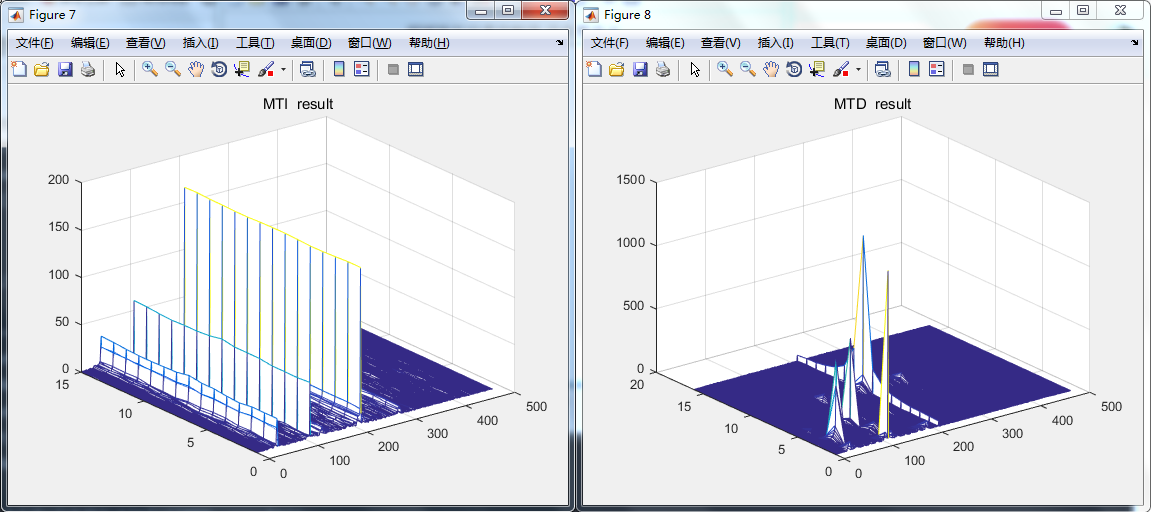6，虚实矩阵转换
coeff_fft_c=zeros(1,2*8192);
for i=1:8192
coeff_fft_c(2*i-1)=real(coeff_fft(i));
coeff_fft_c(2*i)=imag(coeff_fft(i));
end
echo_c=zeros(1,2*TotalNumber);
for i=1:TotalNumber
echo_c(2*i-1)=real(Echo(i));
echo_c(2*i)=imag(Echo(i));
end

function y=maiya(PulseNumber,range,Fs,TargetVelocity,SNR)

C=3e8;
Tr=800e-6;%单脉冲最大可测距离0.5*Tr*C=120000m
Tp=160e-6;
fr=1/Tr;
lamda=0.03;
B=1e6;
%% 产生线性调频信号
number=fix(Fs*Tp);%单脉冲发射信号内采样点数
SampleNumber=fix(Fs*Tr);%一个脉冲采样点数,800；则每个采样间距代表120000/799m
TotalNumber=SampleNumber*PulseNumber;
for i=-fix(number/2):fix(number/2)-1
Chirp(i+fix(number/2)+1)=exp(j*(pi*(B/Tp)*(i/(Fs))^2));
end
figure
plot(real(Chirp));
title('线性调频信号')
coeff=conj(fliplr(Chirp));%匹配滤波函数h
figure
plot(real(coeff))
title('匹配滤波函数h')

W = linspace(-pi,pi,4096);
[h1,w0] = freqz(coeff,1,W);
figure
plot(w0/pi,20*log10(abs(h1/max(h1))));
title('匹配滤波函数H频率响应')
%-------
%% 产生目标回波
TargetDistance(1:2)=range;
DelayNumber(1:2)=fix(Fs*2*TargetDistance(1:2)/C);

TargetFd(1:2)=2*TargetVelocity(1:2)/lamda; %计算目标多卜勒

SignalAll=zeros(1,TotalNumber);%所有脉冲的信号,先填0
for k=1:2% 依次产生各个目标1 2
SignalTemp=zeros(1,SampleNumber);% 一个脉冲

SignalTemp(DelayNumber(k)+1:DelayNumber(k)+number)=(10^(SNR(k)/10))*Chirp;  %一个脉冲的1个目标（未加多普勒速度）
Signal=zeros(1,TotalNumber);
for i=1:PulseNumber
Signal((i-1)*SampleNumber+1:i*SampleNumber)=SignalTemp;
end
FreqMove=exp(j*2*pi*TargetFd(k)*(0:TotalNumber-1)/Fs);%目标的多普勒速度*时间=目标的多普勒相移
Signal=Signal.*FreqMove;
SignalAll=SignalAll+Signal;
end
figure
subplot(2,1,1);plot(real(SignalAll),'r-');title('目标信号的实部');grid on;zoom on;
subplot(2,1,2);plot(imag(SignalAll));title('目标信号的虚部');
grid on;zoom on;
%% 产生噪声
SystemNoise=normrnd(0,10^(-12/10),1,TotalNumber)+j*normrnd(0,10^(-12/10),1,TotalNumber);
%% 回波+噪声
Echo=SignalAll+SystemNoise;   % +SeaClutter+TerraClutter;
for i=1:PulseNumber   %在接收机闭锁期,接收的回波为0
Echo((i-1)*SampleNumber+1:(i-1)*SampleNumber+number)=0;
end
figure
plot(real(Echo));title('总回波信号的实部,闭锁期为0');
%subplot(2,1,2);plot(imag(Echo));title('总回波信号的虚部,闭锁期为0');

%% 回波积累
Echoplus=zeros(1,SampleNumber);
for i=1:PulseNumber
Echoplus1(1:SampleNumber)=Echo((i-1)*SampleNumber+1:i*SampleNumber);
Echoplus=Echoplus+Echoplus1(1:SampleNumber);
end
Echoplus=Echoplus/PulseNumber;
figure
plot(real(Echoplus));title('回波脉冲积累信号的实部,闭锁期为0');

%% 时域脉压
pc_time0=conv(Echo,coeff);
%figure
%plot(abs(pc_time0));title('时域脉压结果的幅度,有暂态点');
%% 频域脉压
Echo_fft=fft(Echo,TotalNumber+number-1);%进行TotalNumber+number-1点FFT
coeff_fft=fft(coeff,TotalNumber+number-1);
pc_fft=Echo_fft.*coeff_fft;
pc_freq0=ifft(pc_fft);
pc_freq_abs=abs(pc_freq0);
figure
plot(pc_freq_abs);title('(不加窗)频域脉压结果的幅度（有暂态点）');

%%  数据重排到距离门
pc_freq1=pc_freq0(number:TotalNumber+number-1);
for i=1:PulseNumber
pc(i,1:SampleNumber)=pc_freq1((i-1)*SampleNumber+1:i*SampleNumber);
end

figure

%subplot(2,1,1)
%plot((0:SampleNumber-1)*(120000/(SampleNumber-1)), abs(pc(5,:)) );
% title('不加窗脉压结果');xlabel('距离'),ylabel('脉压输出');

plot((0:SampleNumber-1)*(120000/(SampleNumber-1)), 20*log10(abs(pc(1,:))) );
xlabel('距离'),ylabel('脉压输出/db');
axis([-inf,inf, -10,50]);
title('不加窗脉压结果')
%% 加窗处理
win=chebwin(number,35)';
h_w=coeff.*win;
hfft_w=fft(h_w,TotalNumber+number-1);
y=abs(ifft(Echo_fft.*hfft_w));
%figure
%plot(y);title('(加窗)频域脉压结果的幅度（有暂态点）');

pcW_freq1=y(number:TotalNumber+number-1);  %重排数据
for i=1:PulseNumber
pcW(i,1:SampleNumber)=pcW_freq1((i-1)*SampleNumber+1:i*SampleNumber);
end

figure
%subplot(2,1,1)
%plot((0:SampleNumber-1)*(120000/(SampleNumber-1)), abs(pcW(1,:)) );
%title('加窗脉压结果');xlabel('距离'),ylabel('脉压输出');

plot((0:SampleNumber-1)*(120000/(SampleNumber-1)), 20*log10(abs(pcW(1,:))) );
xlabel('距离'),ylabel('脉压输出/db');
axis([-inf,inf, -10,50]);
title('加窗脉压结果')

%% 相干积累
Echoplus_fft=fft(Echoplus,SampleNumber+number-1);%理应进行TotalNumber+number-1点FFT,但为了提高运算速度,进行了8192点的FFT
coeffplus_fft=fft(coeff,SampleNumber+number-1);
pcplus_fft=Echoplus_fft.*coeffplus_fft;
pcplus_freq=abs(ifft(pcplus_fft));
%figure
%plot(pcplus_freq);title('(不加窗)积累后频域脉压结果的幅度,有暂态点');

pcplus_freq1=pcplus_freq(number:SampleNumber+number-1);
figure
subplot(2,1,1)
plot((0:SampleNumber-1)*(120000/(SampleNumber-1)), abs(pcplus_freq1) );
title('不加窗积累后脉压结果');xlabel('距离'),ylabel('脉压输出');
subplot(2,1,2)

plot((0:SampleNumber-1)*(120000/(SampleNumber-1)), (20*log10((pcplus_freq1))));
title('不加窗积累后脉压结果');xlabel('距离'),ylabel('脉压输出/DB');
axis([-inf,inf, -20,50]);

%% mti对消
for i=1:PulseNumber-1  %滑动对消，少了一个脉冲 2脉冲
mti(i,:)=pc(i+1,:)-pc(i,:);
end
mti_abs=abs(mti);
figure
mesh(20*log10(mti_abs));title('MTI  result');
xlabel('距离单元');
ylabel('多普勒通道');
zlabel('幅度值');
axis([-inf,inf,-inf,inf, 0,100]);

%% MTD

mtd=zeros(PulseNumber,SampleNumber);
mtd_abs=zeros(PulseNumber,SampleNumber);
for i=1:SampleNumber
buff(1:PulseNumber)=pc(1:PulseNumber,i);
buff_fft=fftshift(fft(buff)); %用fftshift将零频搬移到中间 这样可以方便观察速度正负
mtd(1:PulseNumber,i)=buff_fft(1:PulseNumber)';
end
mtd_abs=abs(mtd);
x=0:1:SampleNumber-1;
y=-PulseNumber/2:1:PulseNumber/2-1;     %通道这样设后读出的通道数乘单位值则是速度值。
figure
mesh(x,y,20*log10(mtd_abs));title('MTD  result');
xlabel('距离单元');
ylabel('多普勒通道');
zlabel('幅度值');
axis([-inf,inf,-inf,inf, 0,100]);

%% cfar

cfar=zeros(PulseNumber,SampleNumber);
T=sqrt(2*(10^(-12/10))^2*log(1/(1e-6)));
for i=1:PulseNumber
for k=1:2
left=0;
right=Mean(mtd_abs(i,:),k+2,k+17);
maxV=max(left,right);
if(mtd_abs(i,k)>=T*maxV)
cfar(i,k)=mtd_abs(i,k);
else
cfar(i,k)=0;
end
end

for k=3:17
left=Mean(mtd_abs(i,:),1,k-2);
right=Mean(mtd_abs(i,:),k+2,k+17);
maxV=max(left,right);
if(mtd_abs(i,k)>=T*maxV)
cfar(i,k)=mtd_abs(i,k);
else
cfar(i,k)=0;
end
end
for k=18:SampleNumber-18
left=Mean(mtd_abs(i,:),k-17,k-2);
right=Mean(mtd_abs(i,:),k+2,k+17);
maxV=max(left,right);
if(mtd_abs(i,k)>=T*maxV)
cfar(i,k)=mtd_abs(i,k);
else
cfar(i,k)=0;
end
end
for k=SampleNumber-17:SampleNumber-2
left=Mean(mtd_abs(i,:),k-17,k-2);
right=Mean(mtd_abs(i,:),k+2,SampleNumber);
maxV=max(left,right);
if(mtd_abs(i,k)>=T*maxV)
cfar(i,k)=mtd_abs(i,k);
else
cfar(i,k)=0;
end
end
for k=SampleNumber-1:SampleNumber
left=Mean(mtd_abs(i,:),k-17,k-2);
right=0;
maxV=max(left,right);
if(mtd_abs(i,k)>=T*maxV)
cfar(i,k)=mtd_abs(i,k);
else
cfar(i,k)=0;
end
end
end
figure

IQ_acc=mean(abs(mti));
mesh(x,y,20*log10(cfar));title(' CFAR  result');
xlabel('距离单元');
ylabel('多普勒通道');
zlabel('幅度值');
axis([-inf,inf,-inf,inf, 0,100]);
figure
plot(x,20*log10(cfar(:,:)),x,20*log10(abs(IQ_acc)));
title(' CFAR  result');
xlabel('距离单元');
ylabel('幅度');
axis([-inf,inf, -20,100]);

展开全文• 雷达领域最经典专著《雷达手册》第三版（中文），第二章，值得收藏，雷达专业必备
• 根据雷达动目标显示、动目标检测原理，通过双脉冲对消，三脉冲对消和盲速处理的方法，用Labview对其进行仿真。仿真结果表明：在动目标显示（MTI）和动目标检测（MTD）中使用滤波器组，能够得到更好的改善因子，降低...
• 这篇文章主要讨论为什么不同脉冲的同一距离门里包含目标的多普勒信息，从原理上进行理解，细节缺乏推敲。 雷达接收信号数据块为： 图片源自wuli huag的博客雷达回波仿真 每一列代表对同一脉冲的采样（快时间），...

这篇文章主要讨论为什么不同脉冲的同一距离门里包含目标的多普勒信息，从原理上进行理解，细节缺乏推敲。
雷达接收信号数据块为：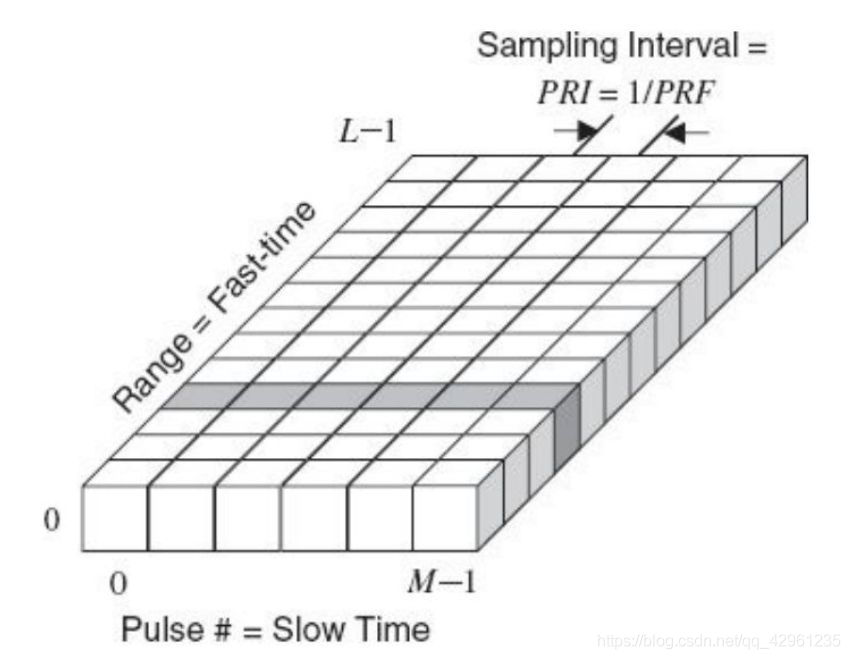图片源自wuli huag的博客雷达回波仿真

每一列代表对同一脉冲的采样（快时间），每一行代表对同一距离门的采样（慢时间）。
对每一列做匹配滤波可以得到目标的距离信息，对每一行做FFT可以得到目标的多普勒信息，也即MTD。
设雷达发射线性调频连续波信号：
s t ( t ) = A 0 e j 2 π f 0 t ∑ m = 0 M − 1 u ( t − m T ) u ( t ) = { e j π K t 2 , 0 ≤ t < T 0 ,   e l s e {{s}_{t}}(t)=A_0{{e}^{j2\pi {{f}_{0}}t}}\sum\limits_{m=0}^{M-1}{u(t-mT)} \\ u(t)=\left\{ \begin{matrix} {{e}^{j\pi K{{t}^{2}}}},0\le t<T \\ 0,\text{ }else \end{matrix} \right.
其中 A 0 A_0 为发射信号的幅度， f 0 f_0 为雷达载频， K K 为调频斜率， T T 为脉冲重复周期， M M 为连续发射的线性调频脉冲数。
设某目标的射线距离为 R R ，径向速度为 v r v_r ，那么该目标的第 m + 1 m+1 个脉冲回波为：
s r ( t ) = s t ( t − t d ) t d = 2 ( R + v r t ) c = 2 R c + f d f 0 t {{s}_{r}}\left( t \right)={{s}_{t}}\left( t-{{t}_{d}} \right) \\ {{t}_{d}}=\frac{2\left( R+{{v}_{r}}t \right)}{c}=\frac{2R}{c}+\frac{{{f}_{d}}}{{{f}_{0}}}t
于是接收信号可以写成：
s r ( t ) = A 0 exp ⁡ ( j 2 π f 0 ( t − t d ) ) ∑ m = 0 M − 1 u ( t − m T − t d ) {{s}_{r}}\left( t \right)=A_0\exp \left( j2\pi {{f}_{0}}\left( t-{{t}_{d}} \right) \right)\sum\limits_{m=0}^{M-1}{u\left( t-mT-{{t}_{d}} \right)}
t d t_d 为时延， f d f_d 为多普勒频移。
先对目标进行距离处理，参考信号取：
s 0 ( t ) = A 0 exp ⁡ ( j 2 π f 0 ( t − τ 0 ) ) u ( t − m T − τ 0 ) {{s}_{0}}\left( t \right)=A_0\exp \left( j2\pi {{f}_{0}}\left( t-{{\tau }_{0}} \right) \right)u\left( t-mT-{{\tau }_{0}} \right)
τ 0 = 2 R c {{\tau }_{0}}=\frac{2R}{c} 为距离 R R 上的时延。
仅关注接收信号的 exp ⁡ ( j 2 π f 0 ( t − t d ) ) \exp \left( j2\pi {{f}_{0}}\left( t-{{t}_{d}} \right) \right) 项，与参考信号混频后剩下 exp ⁡ ( − j 2 π f d t ) \exp \left( -j2\pi {{f}_{d}}t \right) ，可知不同脉冲的同一距离维频率相差 f d T f_dT 。也即对慢时间维做FFT可得到目标的多普勒信息。
下面给出海杂波的仿真实例进行验证。首先是将回波信号匹配滤波后排成行，每一列代表同一距离门，此时的距离-多普勒结果如下图所示，可以看出每一行都是在目标处产生峰值。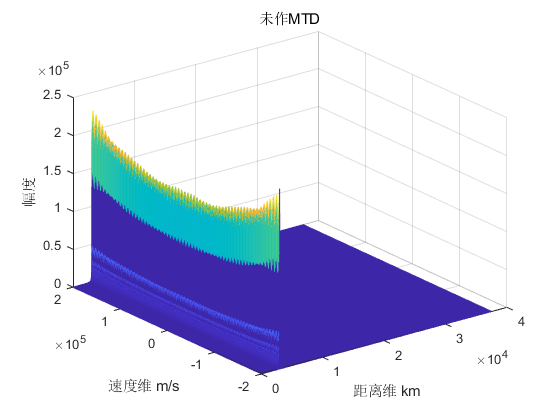对上图的每一列进行FFT，结果如下图所示。虽然看起来只在某一个地方有峰值，我最初有些疑惑，不应该每一列都应该在多普勒频率处有一个峰值吗，其实是这样的，只是由于其他列的峰值与目标所在距离那一列的峰值相比较而言很小，最终呈现的结果只有一个峰值，这样也可以直接读出目标的距离和速度。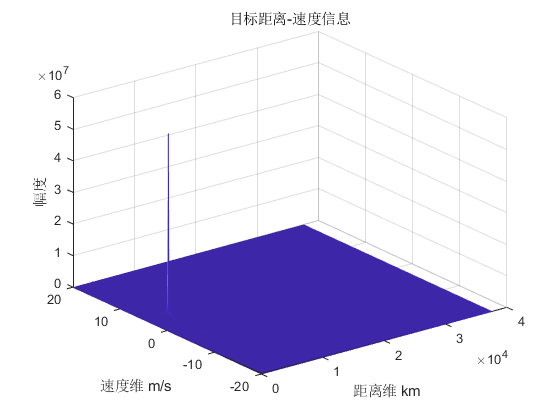目标距离所在列做FFT的结果如下图：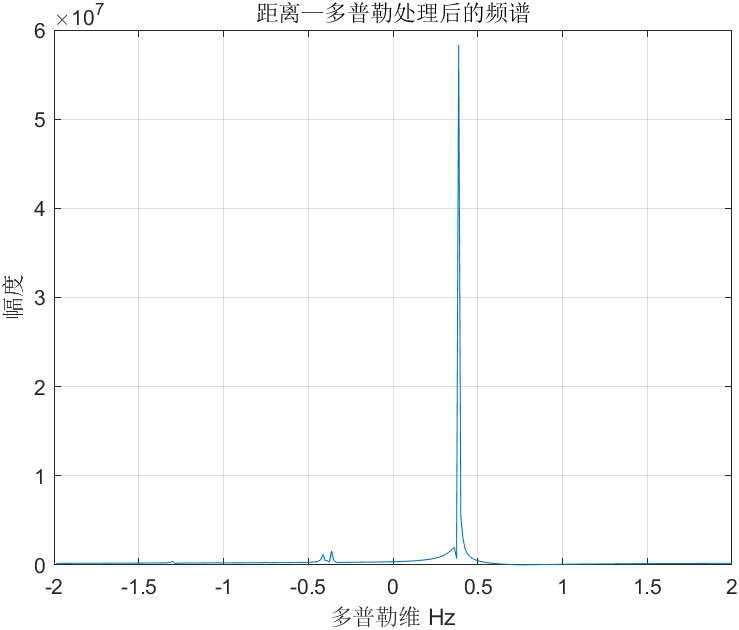为了证明任选一列也可以，这里选择第10列的数据做FFT，结果如下图：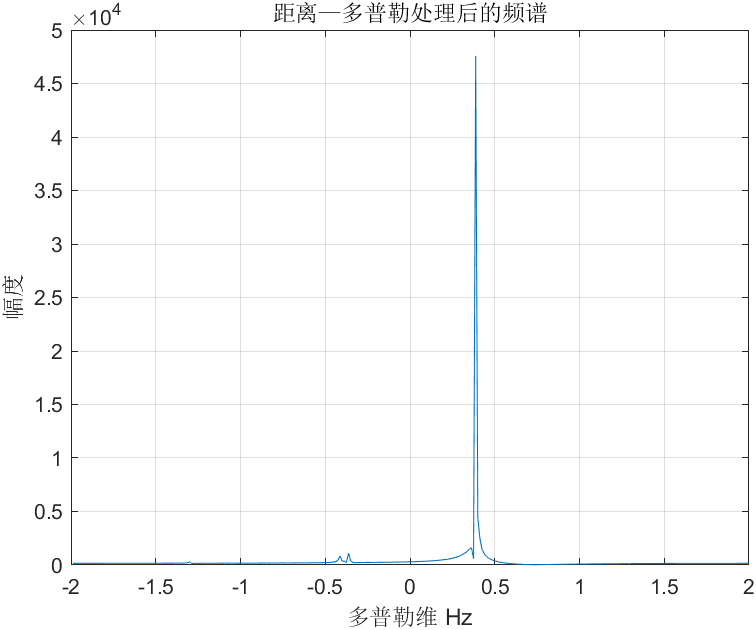从上两幅图的结果可以看出，任选同一距离门数据做FFT即可得到目标速度信息，距离-多普勒图像只有一个峰值的原因在于其他列相对目标距离所在列的峰值小太多，这两幅图分别是 1 0 7 10^7 1 0 4 10^4

参考文献：

1. 郭欣. 天波超视距雷达信号处理技术研究[D].南京理工大学,2004.
2. 戴文娟. 天波超视距雷达海杂波仿真与目标检测算法[D].电子科技大学,2013.
展开全文信号处理
• 动目标指示(MTI)与动目标检测(MTD) 2018-12-10 07:00 多普勒雷达是指利用多普勒效应，测量目标相对于雷达的径向速度分量，或对具有特定径向速度的目标进行提取的雷达。如果雷达发射的是脉冲信号，则称为脉冲多普勒...

转至：https://www.sohu.com/a/280751859_695278
动目标指示(MTI)与动目标检测(MTD)
2018-12-10 07:00
多普勒雷达是指利用多普勒效应，测量目标相对于雷达的径向速度分量，或对具有特定径向速度的目标进行提取的雷达。如果雷达发射的是脉冲信号，则称为脉冲多普勒雷达。

在由地物、海面、云雨、箔条等物体反射所形成的干扰背景(杂波)中，如果目标与杂波的径向速度不同，动目标指示(MTI)雷达或脉冲多普勒(PD)雷达就具有对其进行检测的能力。

在典型民用领域中，为对空中交通实施管制，须具备在强地杂波和气象杂波中对未装载应答机的低空小型飞机进行检测的能力；

在军用领域应用中，可包括对低空飞机和巡航导弹进行检测、也可应用于机载告警与控制系统(AWACS)、机载预警系统(AEW)以及机载拦截雷达等需要在极强的面杂波环境中下视工作的情况。

点击图片可查看相关视频

高重频模式

机载预警系统所采用的多普勒处理技术有三类。第一类是高重频的脉冲多普勒技术，其重频至少为载机与目标临近飞行速度之和所对应多普勒频率的2倍，从而能够产生比较干净的无杂波区，只需采用窄带滤波器即可检测出运动目标。不过这种情况下距离是高度模糊的，通常需要解模糊。相对于低、中重频模式来说，高重频模式的优点在 于可在峰值功率不变的情况下增加所辐射的能量。

利用距离-速度矩阵的每一单元格都包含距离维和速度维(或称为多普勒频率维)信息，前者对应雷达的距离分辨单元，后者则跟波束照射在目标上的驻留时间成反比。

低重频模式

第二类多普勒处理技术称作机载动目标指示技术，这类技术通过天线设计和信号处理消除了载机的运动效应。一旦消除这种影响，就可采用跟陆基或海基MTI雷达同样的信号处理方式了。由于希望距离是不模糊的，因此这种机载预警雷达经常釆用低重频工作，而对于低重频模式所导致的目标“盲速”问题，可采用重频参差加以解决。

中重频模式

第三类机载预警雷达采用的是中重频模式，此时距离和速度都是模糊的。高于低重频的 主要原因是为了提高对抗主瓣杂波和地面运动目标的能力；而低于高重频则是使雷达具备检 测副瓣杂波中速度较低（甚至为负值）的临近飞行目标的能力。

PRF 优点 缺点

•没有距离模糊

•精确距离测量

•距离分辨率高

•通过距离选通进行旁瓣杂波抑制

•处理简单

•盲速的存在

•高的多普勒模糊

•下视模式时，检测性能低

•高峰值功率或需要距离压缩

•宽范围内目标多普勒的良好检测

•有效抑制主瓣杂波及旁瓣杂波

•精确测距

•相对于高PRF操作,遮蔽降低

•所有速度上存在旁瓣杂波

•PRF和脉冲宽度的乘积大

•复杂的距离多普勒解模糊处理

•对旁瓣中大目标的检测性能低

•高的平均功率

•无模糊多普勒

•没有盲速

•主瓣杂波抑制的同时不会抑制目标

•高模糊距离

•目标遮蔽增加

•测距复杂且精度降低

•由于旁瓣杂波,对低多普勒目标的敏感度降低

运动目标指示(MTI)

由一个CPI内的连续M个脉冲回波经过相干解调后的基带数据，形成的一个二维数据矩阵。二维数据矩阵中的每一列都对应于对一个脉冲回波的连续釆样，即连续的距离单元。列中的每一个元素都是一个复数，代表一个距离单元的实部和虚部(I和Q)分量。因此，二维数据矩阵中的每一行代表对同一距离单元的一连 串脉冲测量。

MTI处理器对慢时间数据序列执行线性滤波处理，以抑制数据中的杂波分量。下图描述了MTI的处理过程。MTI处理所需要的滤波器类型也可以由下图来理解。

上述MTI处理中只能给出在感兴趣的距离单元中是否存在目标的判决信息，并没有提供任何关于目标多普勒频率的估计信息。

动目标检测(MTD)

MTD与MTI处理不同，它直接对每一个距离单元内的慢时间数据序列执行谱分析从而替代滤波处理。目标检测直接在距离-多普勒矩阵数据上进行。

通过计算每个距离单元慢时间信号的一维频谱，从快时间-慢时间CPI矩阵中得到它。最常用的谱分析方法是计算数据矩阵中每一行慢时间数据序列的离散傅里叶变换(DFT)，也可以采用其他谱分析方法。

如果DFT输出中的某些峰值远远高出噪声电平，而且超过一个适当的检测阈值，则认为这些峰值是运动目标的响应，但不能保证DFT采样精确落在峰值位置。

因此，DFT采样的幅度和频率估计仅仅是峰值实际幅度和频率的近似。可以通过内插等方式提高对真实多普勒频率的估计精度。当然，其他现代谱估计方法也可以用于脉冲多普勒处理。

MTI和MTD级联

考虑到杂波通常是最强的信号分量，它可以超出目标信号几十dB。如果在MTI滤波之前就计算慢时间信号的DFT，直流附近杂波响应的旁瓣可能会淹没附近速度的潜在目标响应，从而导致这些目标无法被检测到。

通常，MTI滤波器通常放在前面，利用MTI滤波处理进行总的杂波抑制，再利用脉冲多普勒谱分析对脉冲多普勒谱进行详细检测(MTD)。

欢迎使用Markdown编辑器

你好！ 这是你第一次使用 Markdown编辑器 所展示的欢迎页。如果你想学习如何使用Markdown编辑器, 可以仔细阅读这篇文章，了解一下Markdown的基本语法知识。

新的改变

我们对Markdown编辑器进行了一些功能拓展与语法支持，除了标准的Markdown编辑器功能，我们增加了如下几点新功能，帮助你用它写博客：

1. 全新的界面设计 ，将会带来全新的写作体验；
2. 在创作中心设置你喜爱的代码高亮样式，Markdown 将代码片显示选择的高亮样式 进行展示；
3. 增加了 图片拖拽 功能，你可以将本地的图片直接拖拽到编辑区域直接展示；
4. 全新的 KaTeX数学公式 语法；
5. 增加了支持甘特图的mermaid语法1 功能；
6. 增加了 多屏幕编辑 Markdown文章功能；
7. 增加了 焦点写作模式、预览模式、简洁写作模式、左右区域同步滚轮设置 等功能，功能按钮位于编辑区域与预览区域中间；
8. 增加了 检查列表 功能。

功能快捷键

撤销：Ctrl/Command + Z
重做：Ctrl/Command + Y
加粗：Ctrl/Command + B
斜体：Ctrl/Command + I
标题：Ctrl/Command + Shift + H
无序列表：Ctrl/Command + Shift + U
有序列表：Ctrl/Command + Shift + O
检查列表：Ctrl/Command + Shift + C
插入代码：Ctrl/Command + Shift + K
插入链接：Ctrl/Command + Shift + L
插入图片：Ctrl/Command + Shift + G
查找：Ctrl/Command + F
替换：Ctrl/Command + G

合理的创建标题，有助于目录的生成

直接输入1次#，并按下space后，将生成1级标题。
输入2次#，并按下space后，将生成2级标题。
以此类推，我们支持6级标题。有助于使用TOC语法后生成一个完美的目录。

如何改变文本的样式

强调文本 强调文本

加粗文本 加粗文本

标记文本

删除文本

引用文本

H2O is是液体。

210 运算结果是 1024.

插入链接与图片

图片:带尺寸的图片:居中的图片:居中并且带尺寸的图片:当然，我们为了让用户更加便捷，我们增加了图片拖拽功能。

如何插入一段漂亮的代码片

博客设置页面，选择一款你喜欢的代码片高亮样式，下面展示同样高亮的 代码片.

// An highlighted block
var foo = 'bar';

• 项目
• 项目
• 项目
1. 项目1
2. 项目2
3. 项目3
• 计划任务
• 完成任务

创建一个表格

一个简单的表格是这么创建的：

项目Value
电脑$1600 手机$12
导管\$1

设定内容居中、居左、居右

使用:---------:居中
使用:----------居左
使用----------:居右

第一列第二列第三列
第一列文本居中第二列文本居右第三列文本居左

SmartyPants

SmartyPants将ASCII标点字符转换为“智能”印刷标点HTML实体。例如：

TYPEASCIIHTML
Single backticks'Isn't this fun?'‘Isn’t this fun?’
Quotes"Isn't this fun?"“Isn’t this fun?”
Dashes-- is en-dash, --- is em-dash– is en-dash, — is em-dash

创建一个自定义列表

Markdown
Text-to- HTML conversion tool
Authors
John
Luke

一个具有注脚的文本。2

注释也是必不可少的

Markdown将文本转换为 HTML

KaTeX数学公式

您可以使用渲染LaTeX数学表达式 KaTeX:

Gamma公式展示 Γ ( n ) = ( n − 1 ) ! ∀ n ∈ N \Gamma(n) = (n-1)!\quad\forall n\in\mathbb N 是通过欧拉积分

Γ ( z ) = ∫ 0 ∞ t z − 1 e − t d t   . \Gamma(z) = \int_0^\infty t^{z-1}e^{-t}dt\,.

你可以找到更多关于的信息 LaTeX 数学表达式here.

新的甘特图功能，丰富你的文章

• 关于 甘特图 语法，参考 这儿,

UML 图表

可以使用UML图表进行渲染。 Mermaid. 例如下面产生的一个序列图：

这将产生一个流程图。:

• 关于 Mermaid 语法，参考 这儿,

FLowchart流程图

我们依旧会支持flowchart的流程图：

• 关于 Flowchart流程图 语法，参考 这儿.

导出与导入

导出

如果你想尝试使用此编辑器, 你可以在此篇文章任意编辑。当你完成了一篇文章的写作, 在上方工具栏找到 文章导出 ，生成一个.md文件或者.html文件进行本地保存。

导入

如果你想加载一篇你写过的.md文件，在上方工具栏可以选择导入功能进行对应扩展名的文件导入，
继续你的创作。

1. 注脚的解释 ↩︎

展开全文• 雷达原理-运动目标检测与测速

千次阅读 多人点赞 2020-07-10 18:12:24
文章目录多普勒效应及其应用动目标显示雷达盲速、盲相的影响及其解决途径回波和杂波的频谱及动目标显示滤波器动目标检测(MTD) 多普勒效应及其应用 多普勒效应 连续波雷达 连续波雷达收发天线不共用 脉冲雷达...

本篇博文是看完西安电子科技大学的魏青老师的课程所做的学习笔记，特此记录。

多普勒效应及其应用

1. 多普勒效应
1. 连续波雷达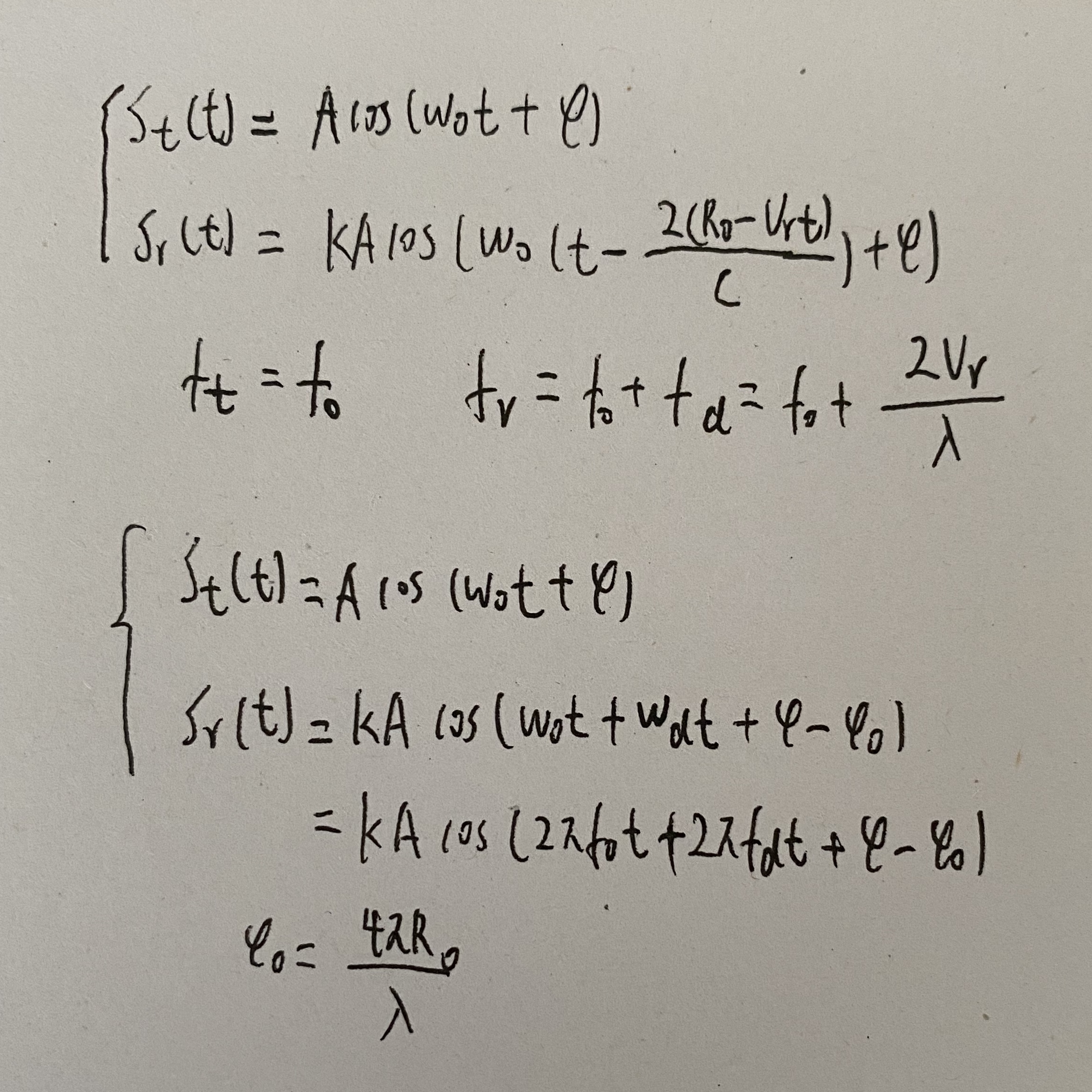连续波雷达收发天线不共用
2. 脉冲雷达（脉冲多普勒雷达）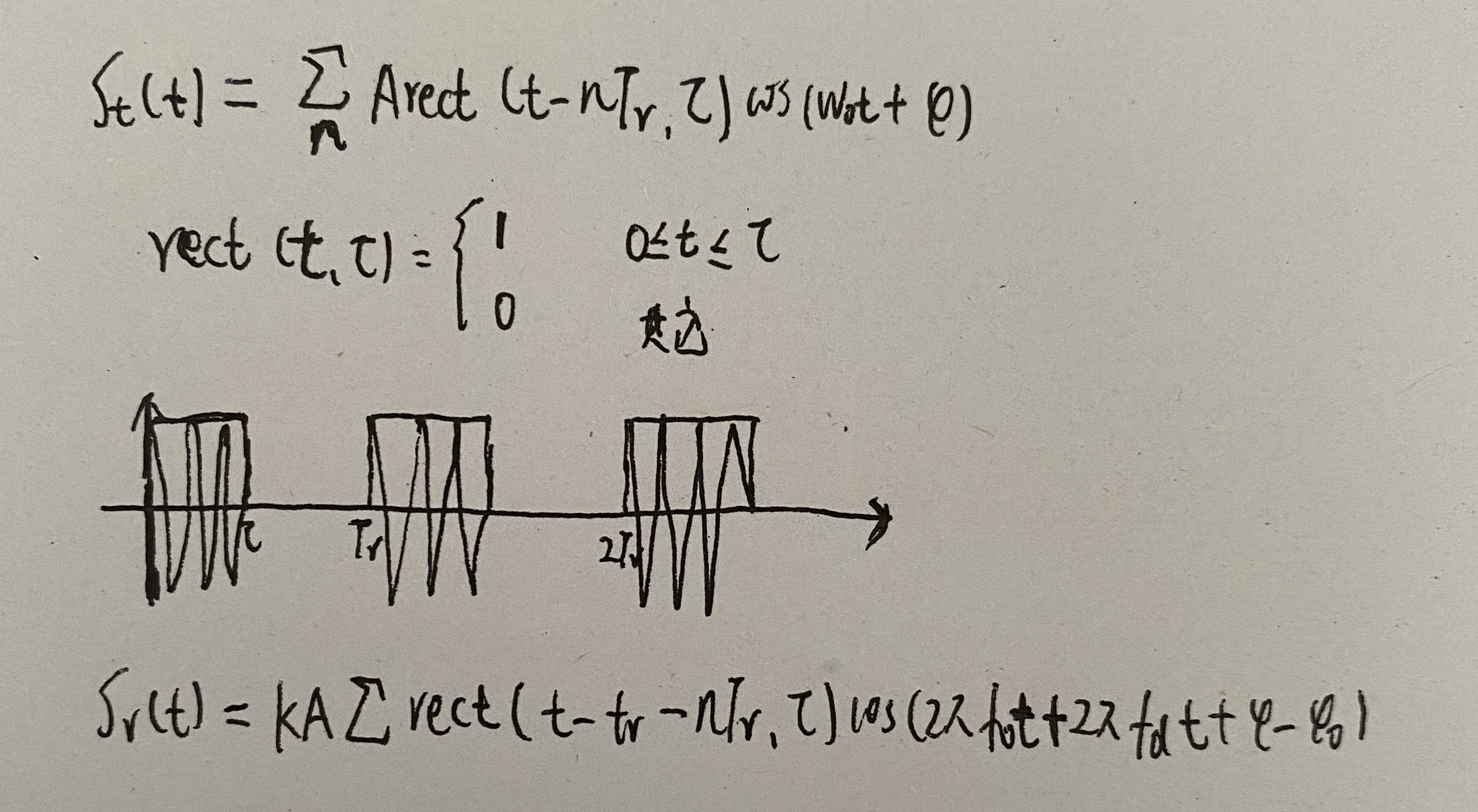2. 多普勒信息的提取
1. 连续波雷达
相位检波器：将发射的信号与接收到的信号进行混频，取低通。
连续波多普勒雷达的组成方框图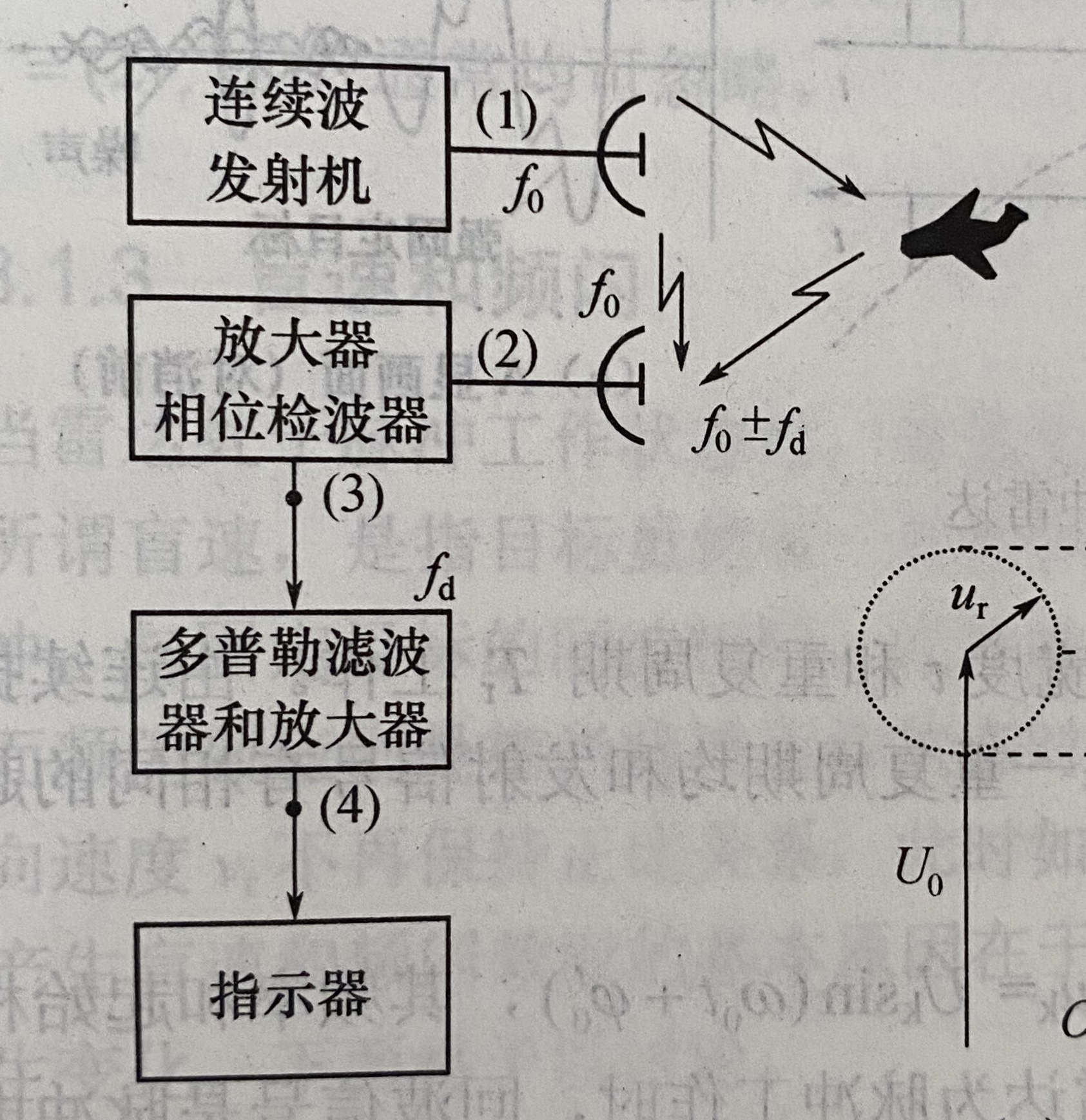通过相位检波器得到的多普勒频率信号为：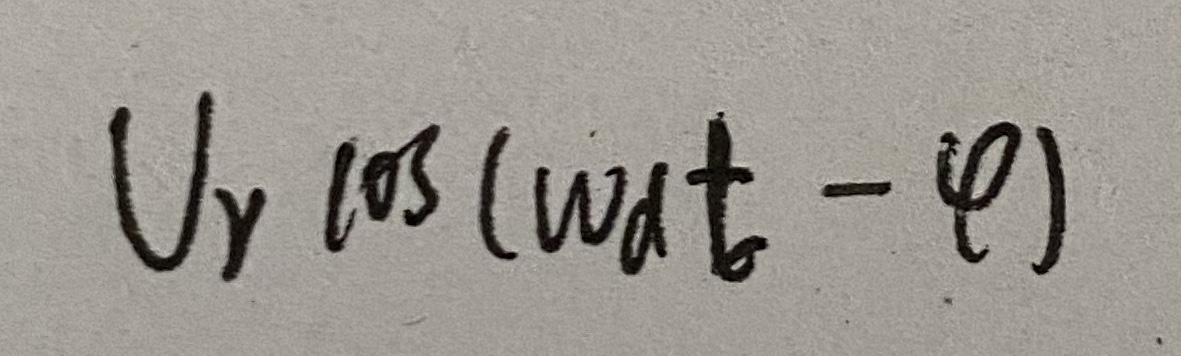2. 脉冲雷达
多普勒效应的脉冲雷达的原理方块图和主要波形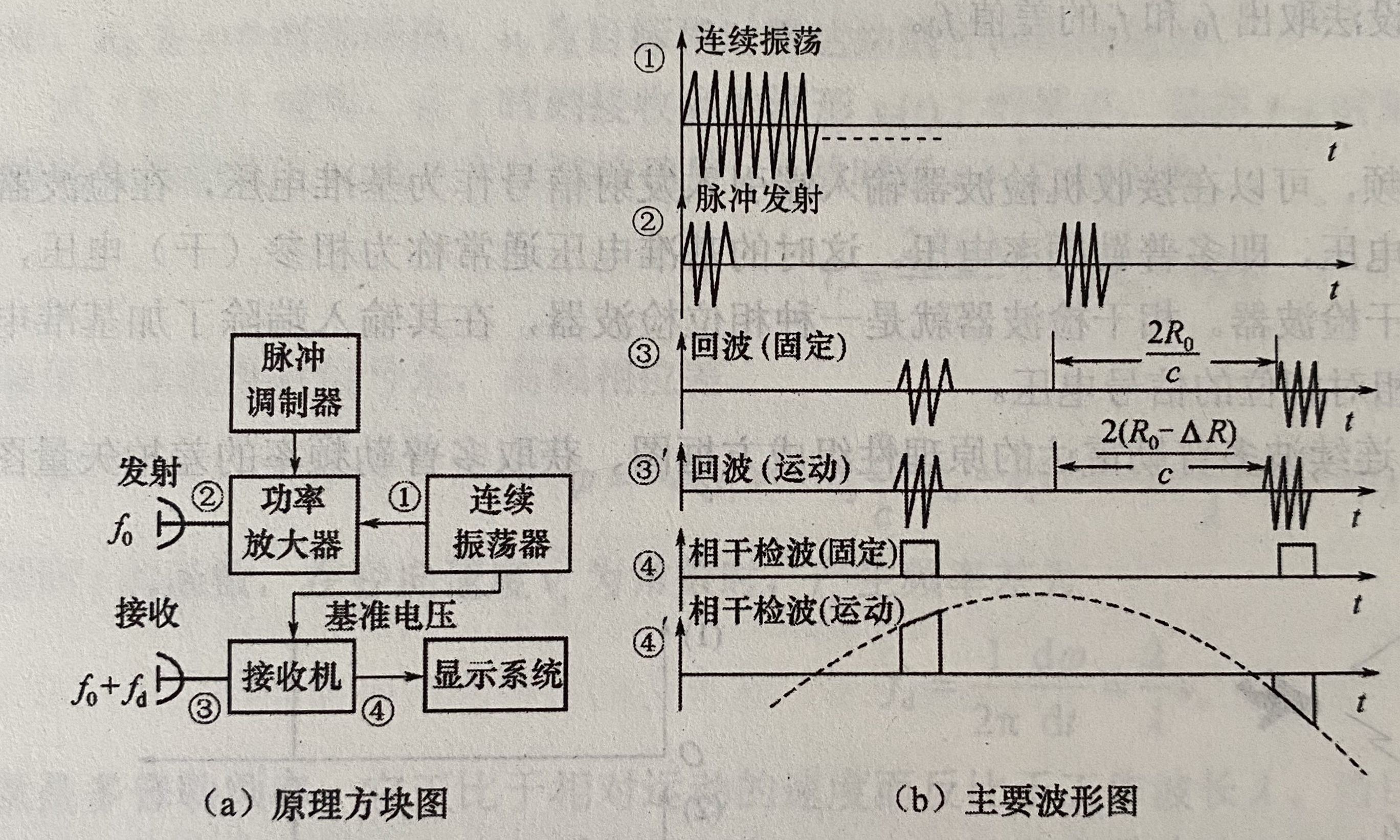3. 盲速和频闪
1. 盲速
盲速是指当目标虽然有一定的径向速度vr，但若其回波信号经过相位检波器后，输出为一串等幅脉冲，与固定目标的回波相同，此时的目标速度为盲速
盲速产生的原因：当fd=nfr时，运动目标回波的谱线由nfr组成，频谱结构与固定目标回波的相同，这是无法区分运动目标与固定目标
盲速说明：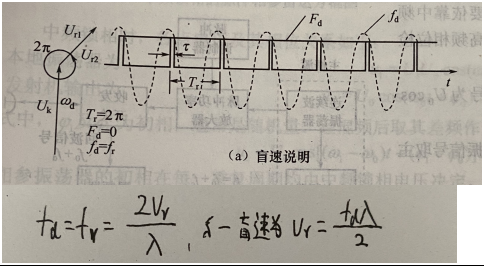2. 频闪
频闪说明：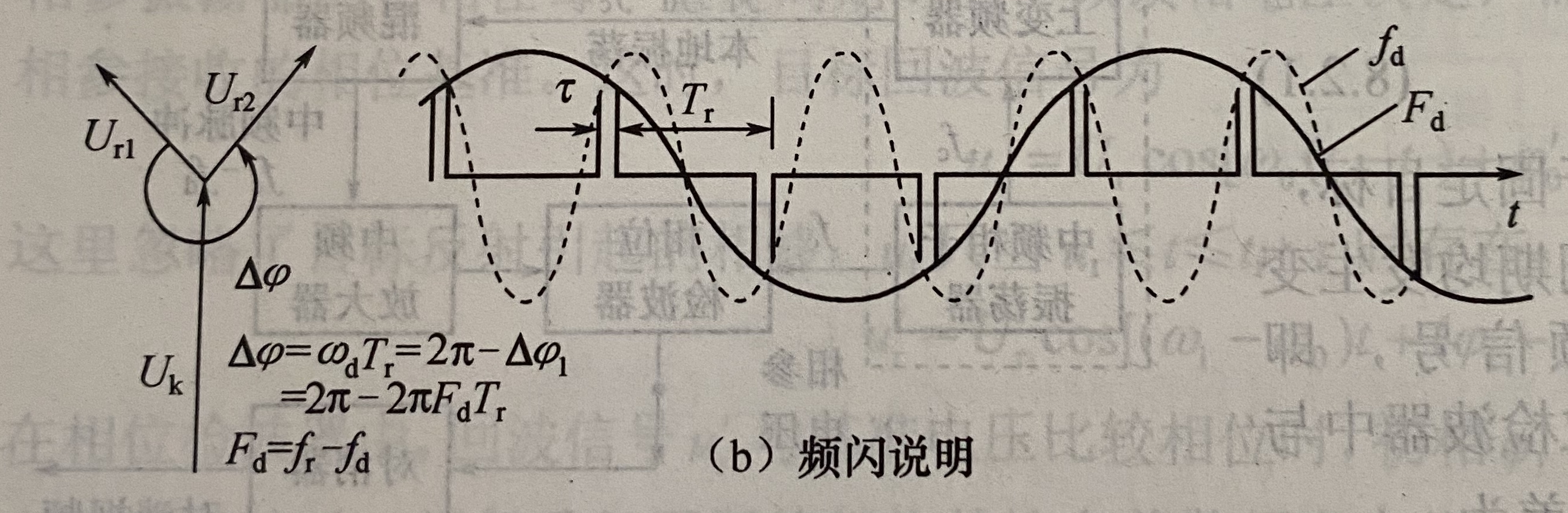频闪是指当脉冲工作状态时，相位检波器输出端回波脉冲串的包络调制频率Fd与目标运动的径向速度vr不再保持正比例关系，此时如用包络调制频率测速时将产生测速模糊。
频闪产生的原因：当多普勒频率fd超过重复频率fr一半时，频率nfr的上边频分量nfr+fd与频率(n+1)fr-fd在谱线排列的前后位置上交叉。两个不同的多普勒频率fd1和fd2，只要满足fd1=nfr-fd2，则二者的谱线位置相同而无法区分。
避免频闪：|fd|<=fr/2

动目标显示雷达

1. 基本工作原理
经过相捡后，去除固定目标回波
2. 中频部分进行相检原理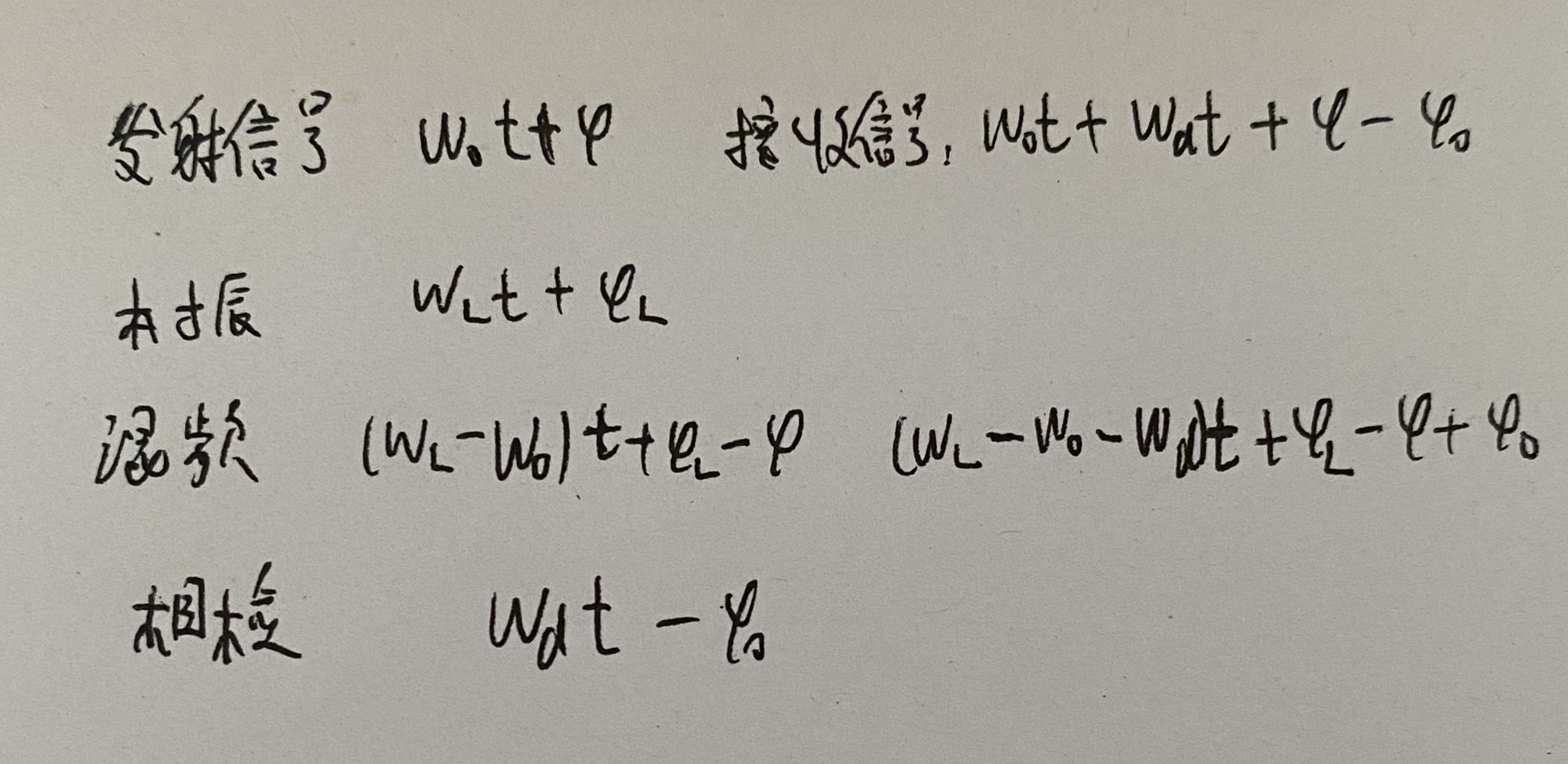3. 消除固定目标回波
一次相消器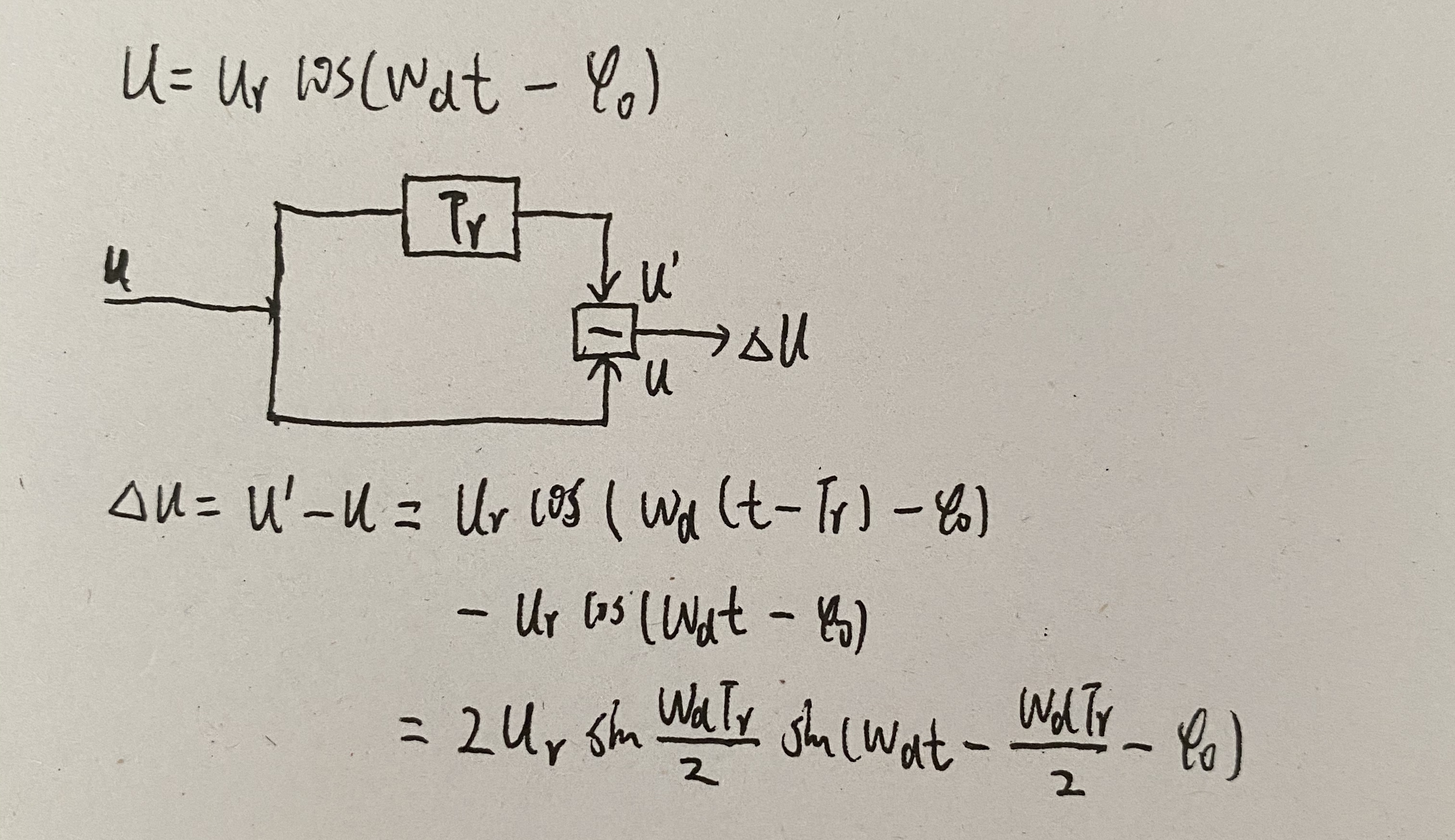当wdTr/2=nπ(n=1，2，3，……)时，输出振幅为零。这时的目标速度相当于盲速。此时，运动目标回波在相位检波器的输出端与固定目标的回波相同，因此经过相消设备后输出为零。
求解相消设备的频率相应特性：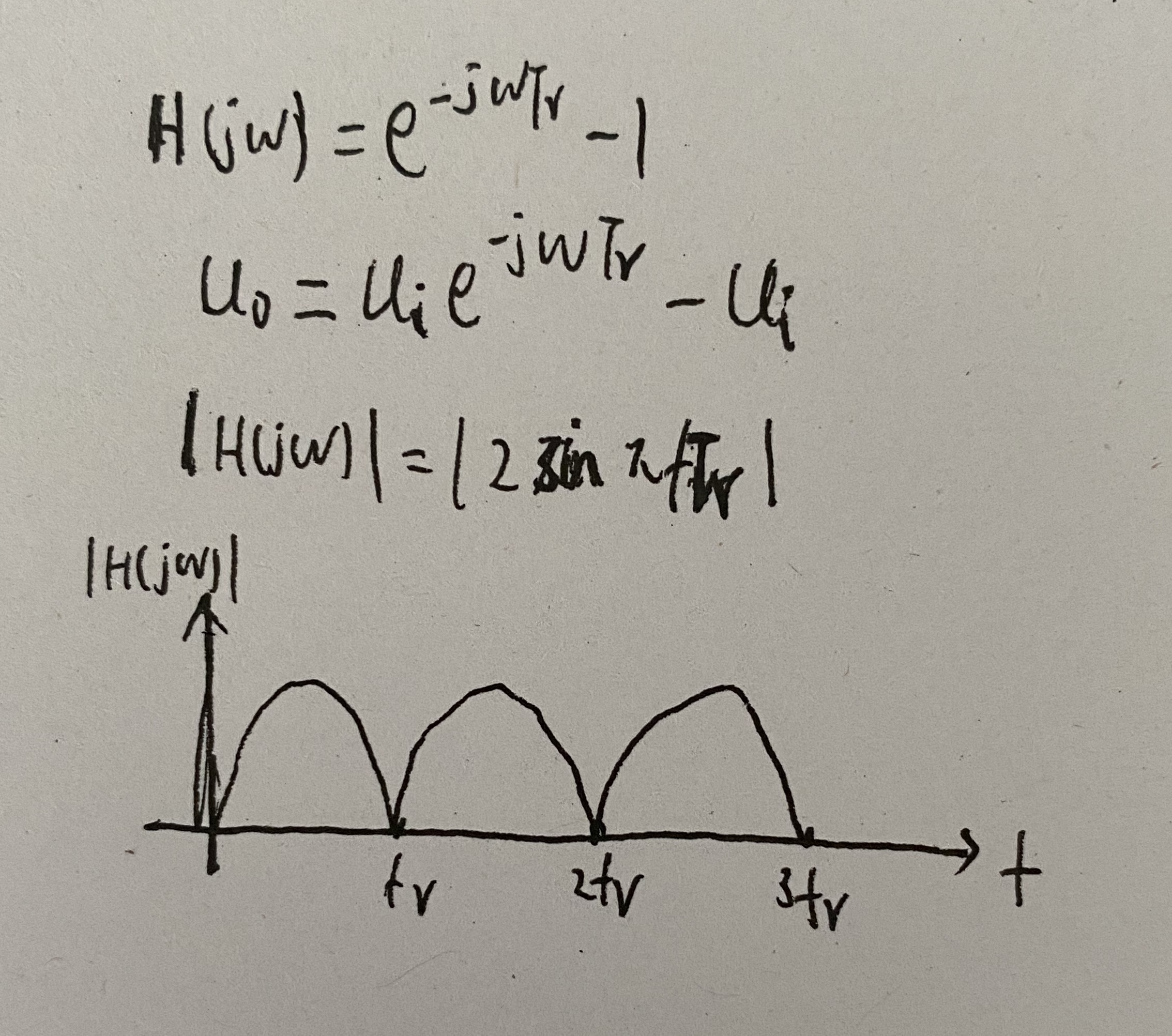盲速、盲相的影响及其解决途径

1. 盲速
1. 盲速出现的条件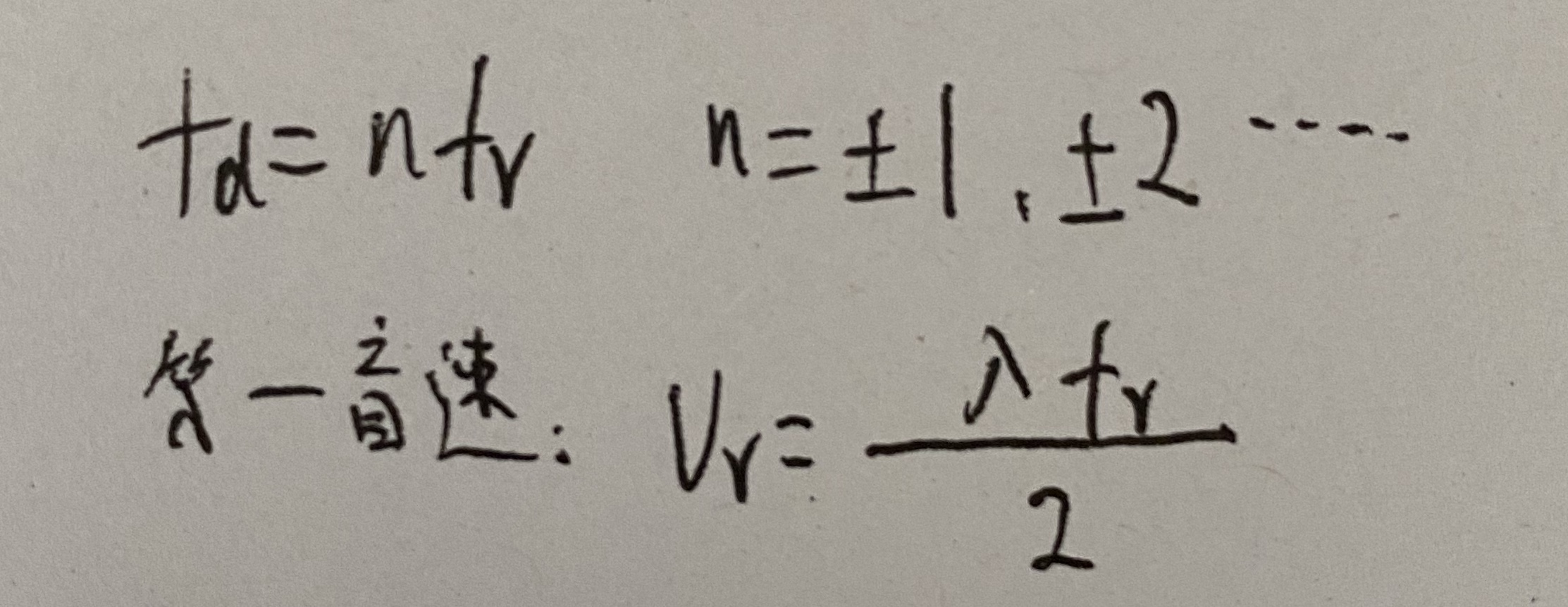最大不模糊距离R0max和第一盲速vr0’的关系: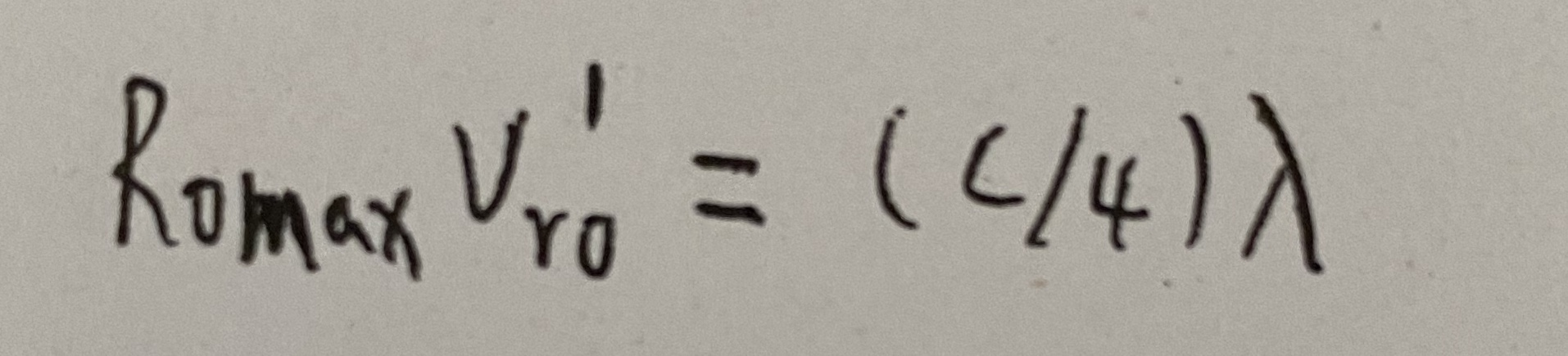2. 重频参差
fd=n*(fr1与fr2的最小公倍数)
2. 盲相
1. 分类
盲相问题是由检波器特性所引起的
相位检波器输出经一次相消器后，运动目标回波为：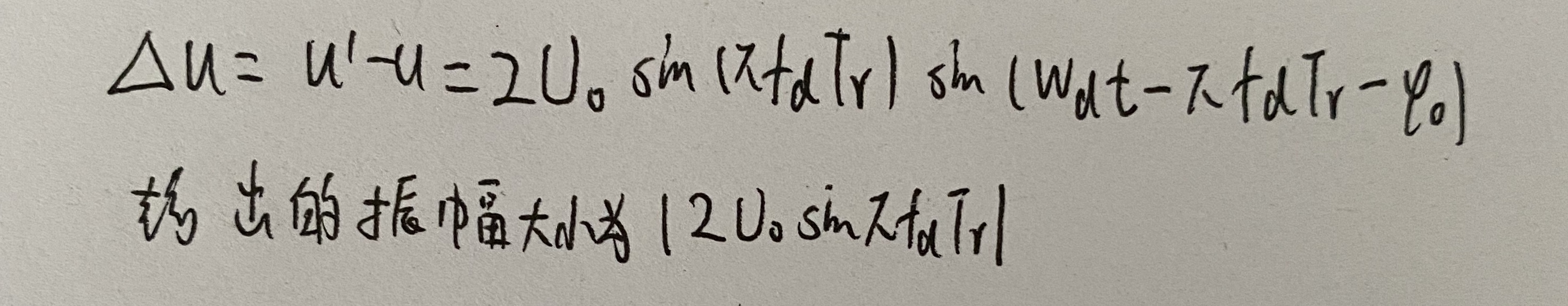在某些点上，输出幅度为零，这些点称为盲相，它由相位检波器的特性决定。从相检特性上看，如果相邻两个回波的脉冲的相位相当于相检特性的a、c两点，其相位差虽不同，但却是一对相检器输出相等的工作点，因此，经过相消器后，其输出为零而出现盲相
相检特性和相消器输出脉冲波形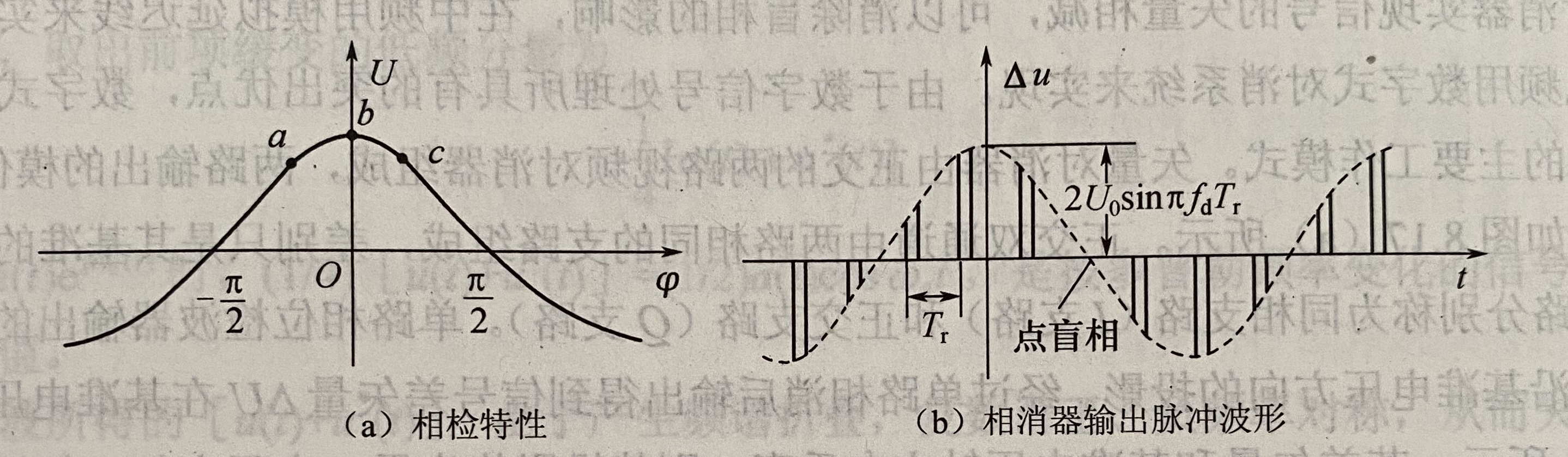用矢量图来说明相消器的输出。匀速运动目标的回波信号用围绕基准电压均匀旋转的一个矢量来表示，旋转的速度等于其多普勒频率。相检器的输出为该矢量沿基准电压方向的投影，如下图所示，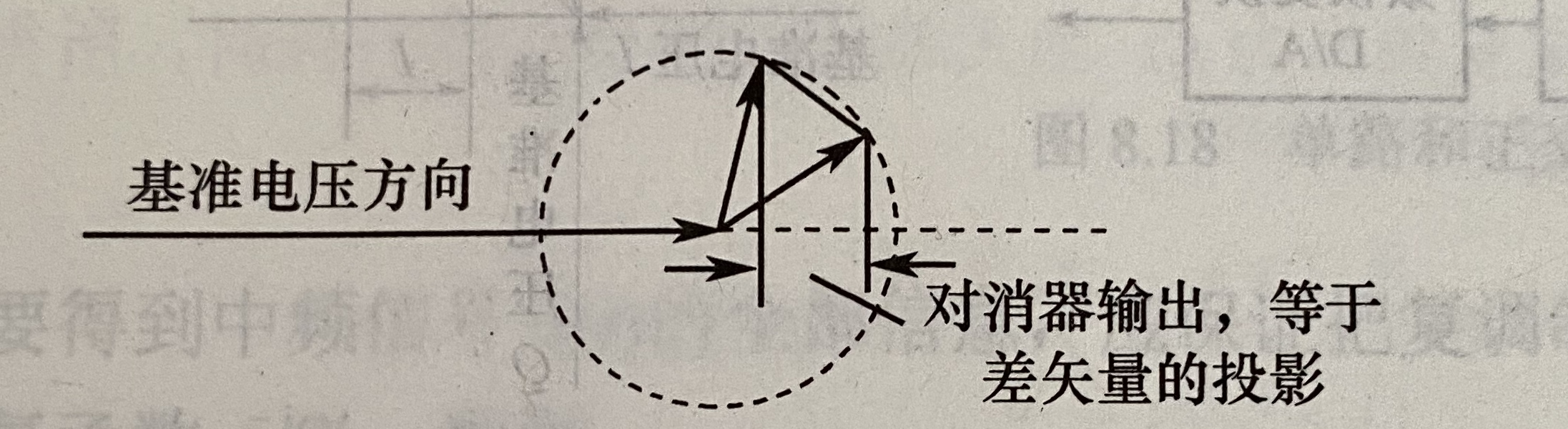当差矢量垂直于该轴时，投影长度为零而出现点盲相
下图表示动目标与固定目标叠加：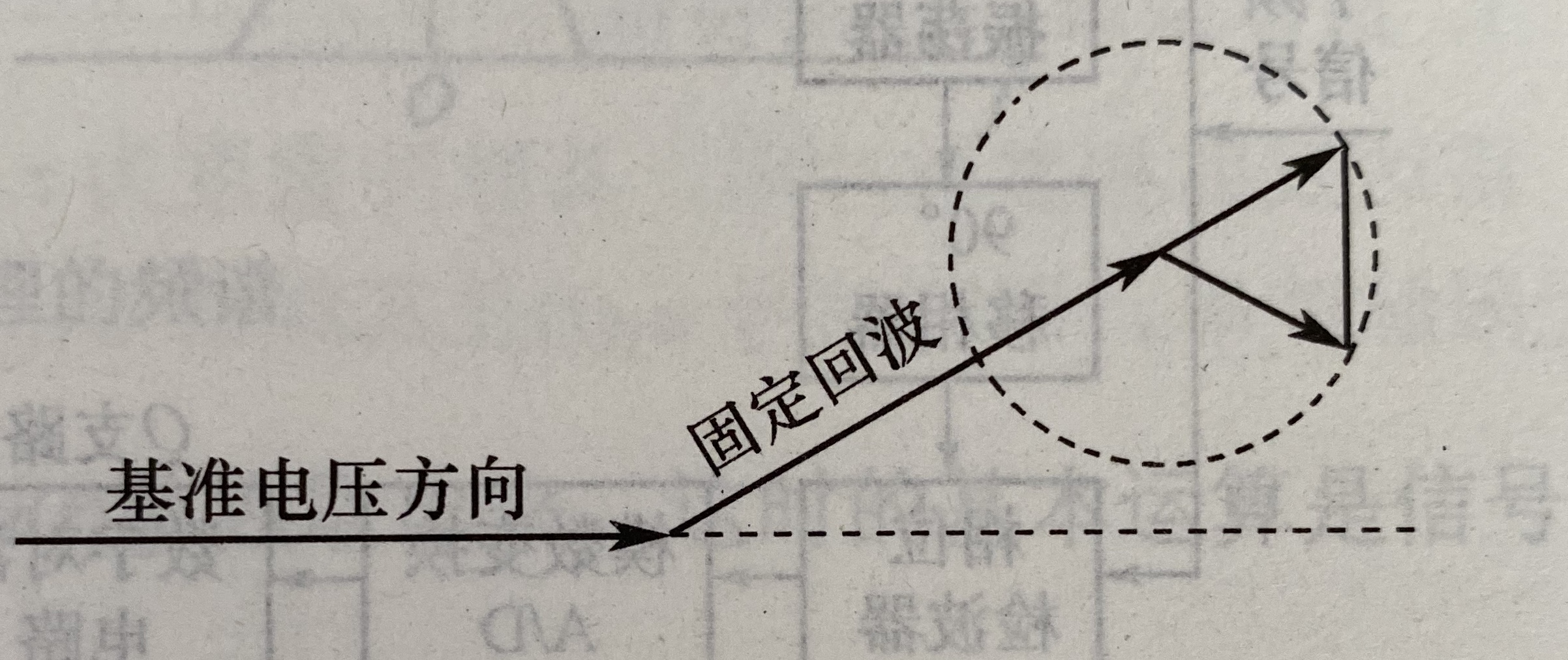如果运动目标回波叠加在固定杂波上，则在一般情况下也将产生点盲相。
如果回波叠加在很强的杂波上，可能产生连续盲相：接收机的限幅作用使动目标和固定杂波的合成矢量变成端点在限幅电平的一小段圆弧上来回摆动的矢量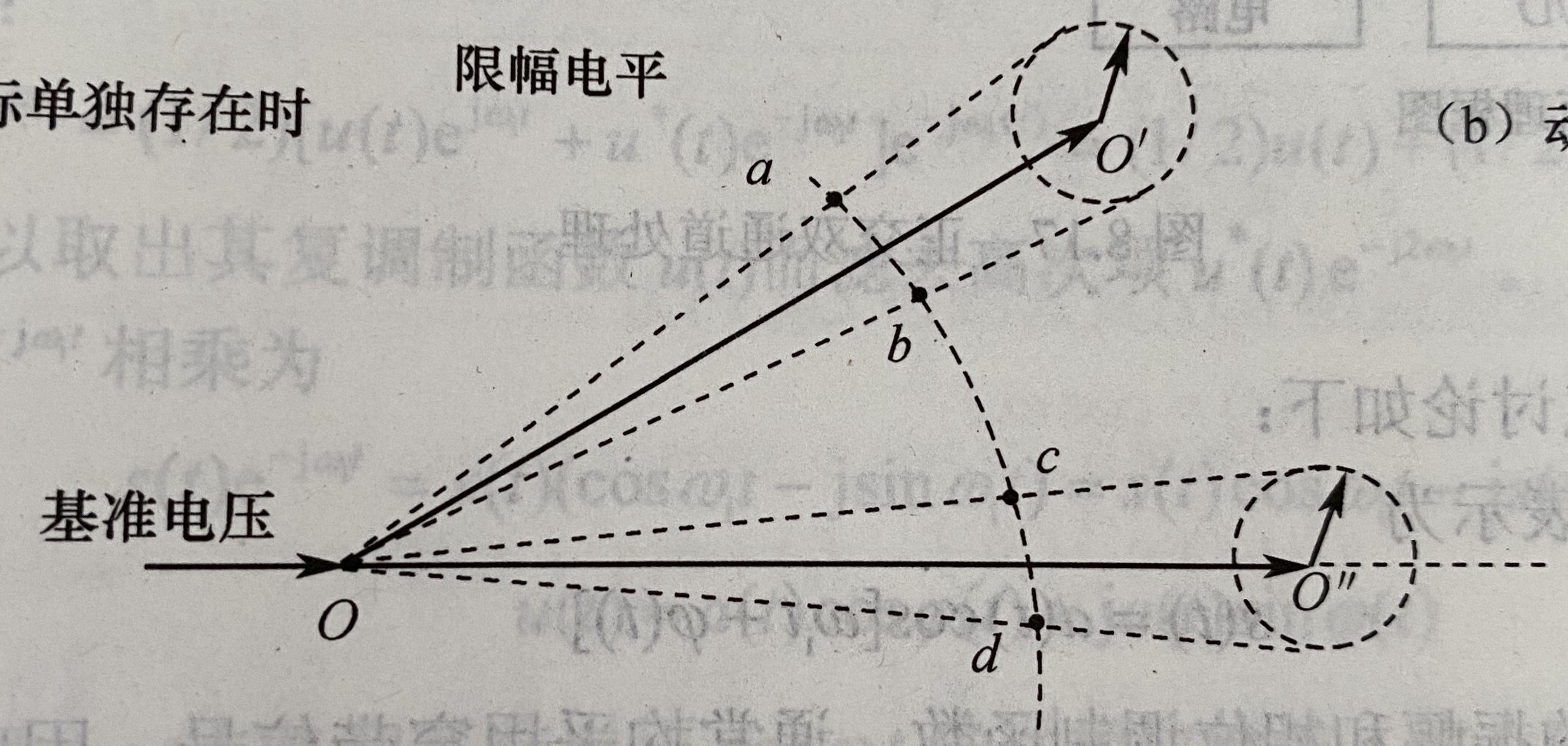2. 解决办法
1. 中频对消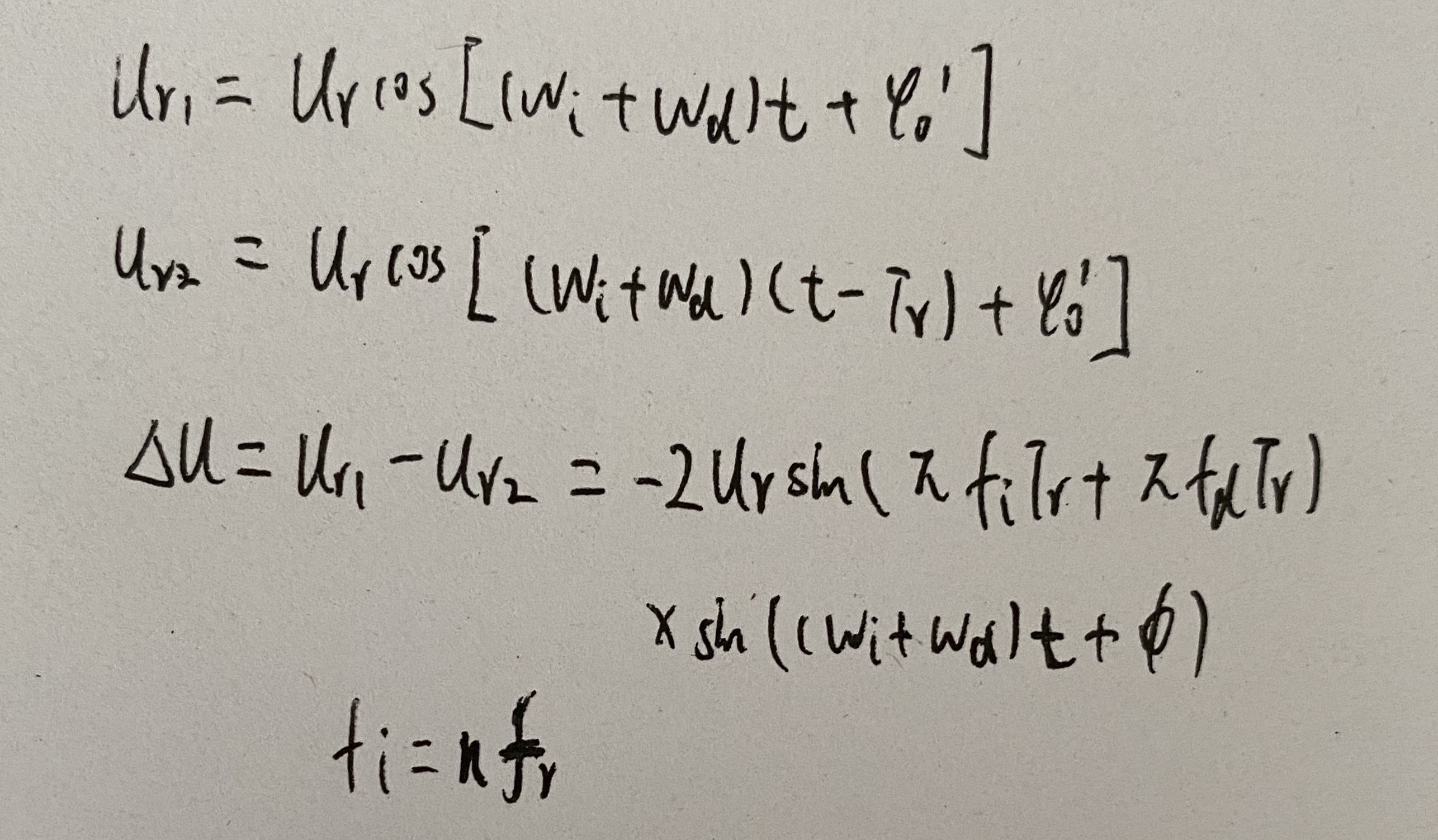2. 零中频（I、Q双通道处理）
原理框图：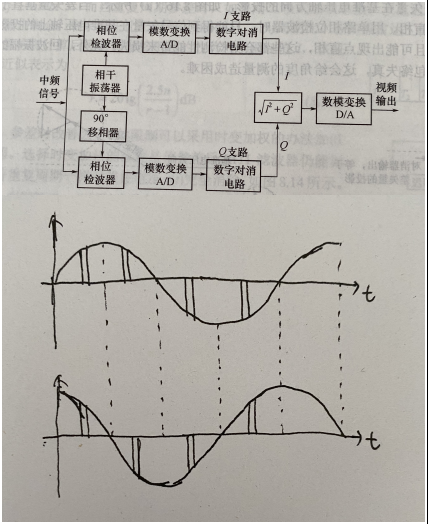用正交双通道，则二路可以同时得到两个投影I和Q，它们互相正交，当一个为零时，另一个值最大。

回波和杂波的频谱及动目标显示滤波器

1. 回波信号和杂波的频谱
1. 雷达回波信号的频谱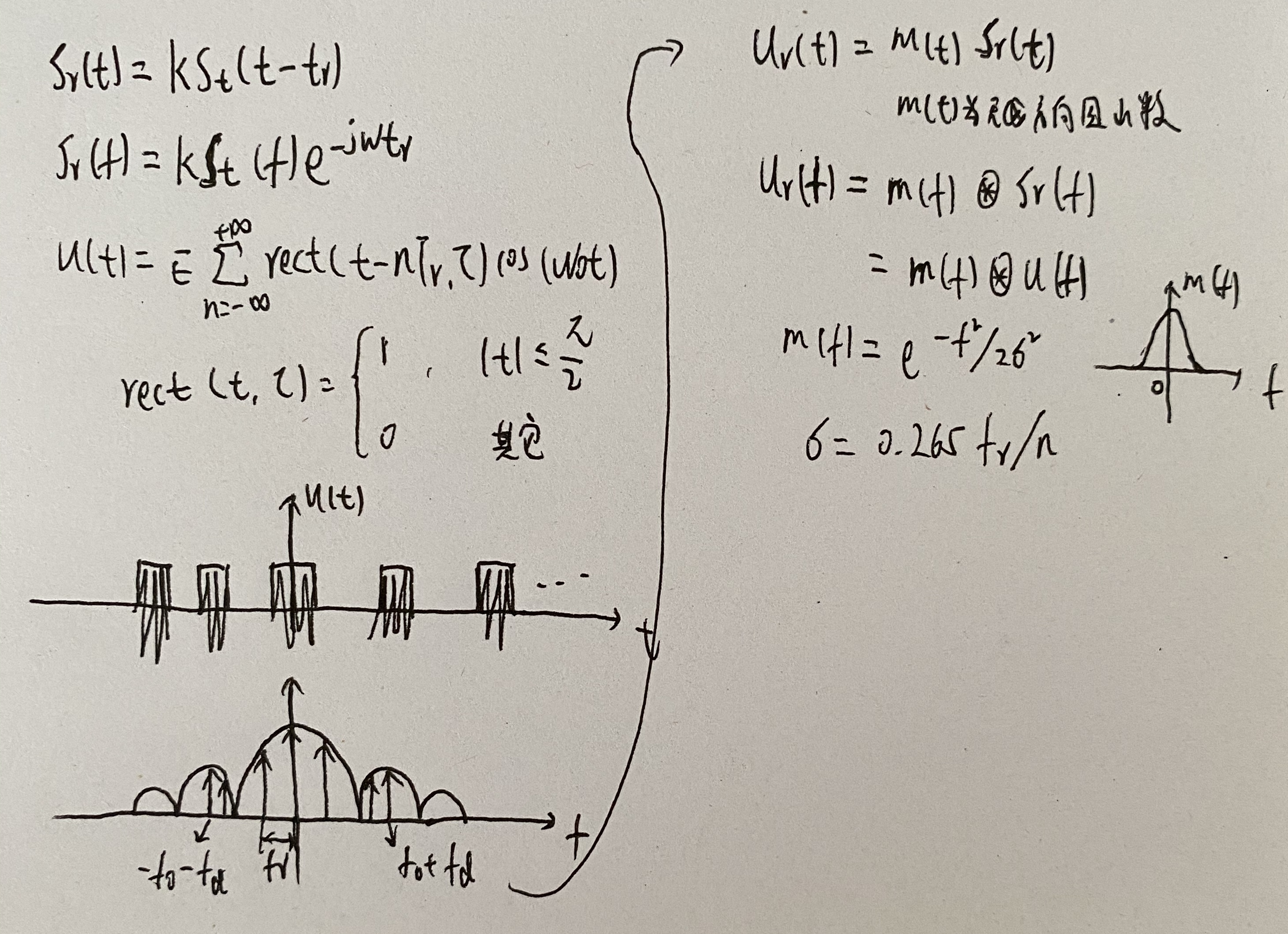天线扫描条件下的回波信号谱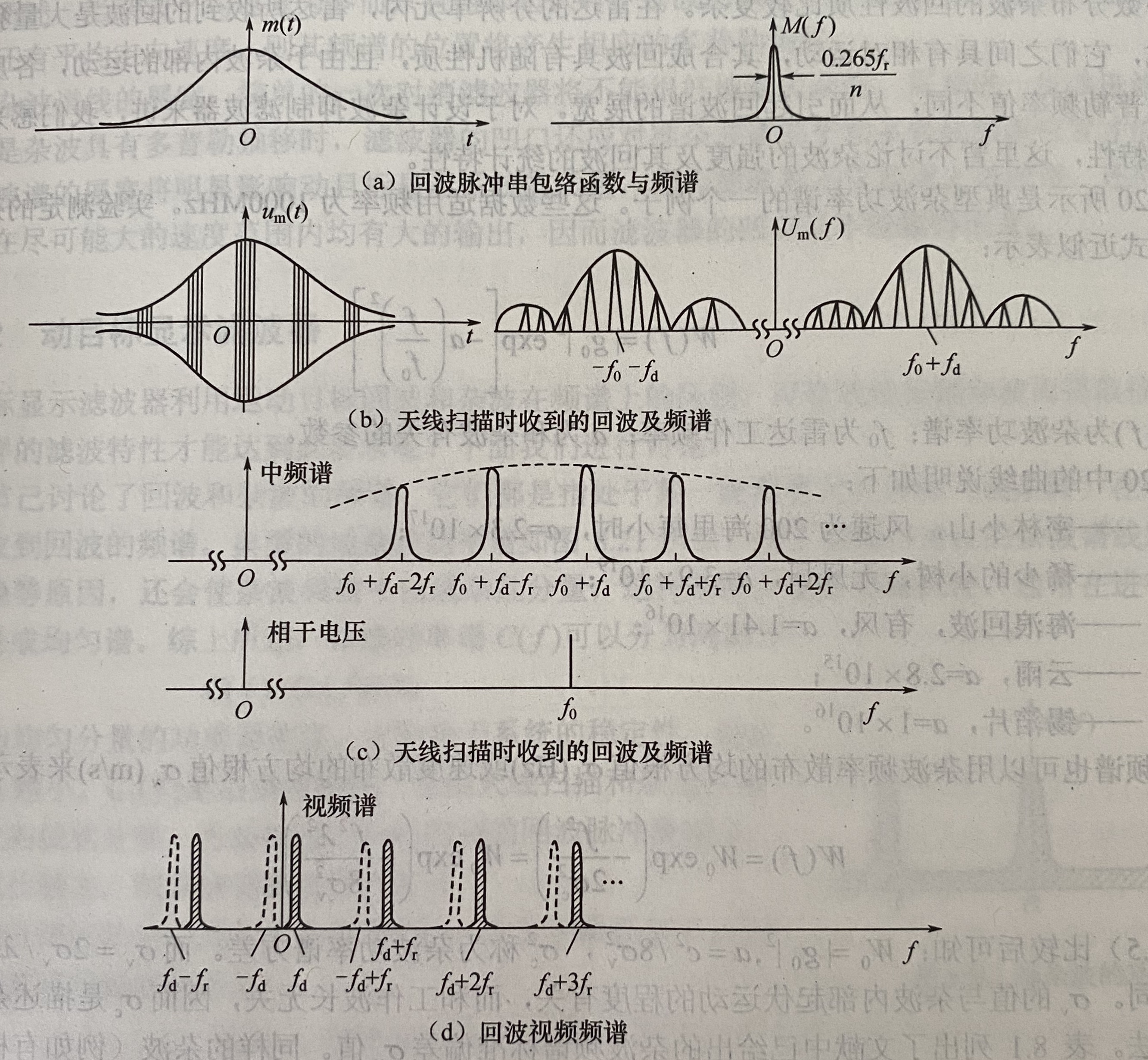2. 杂波频谱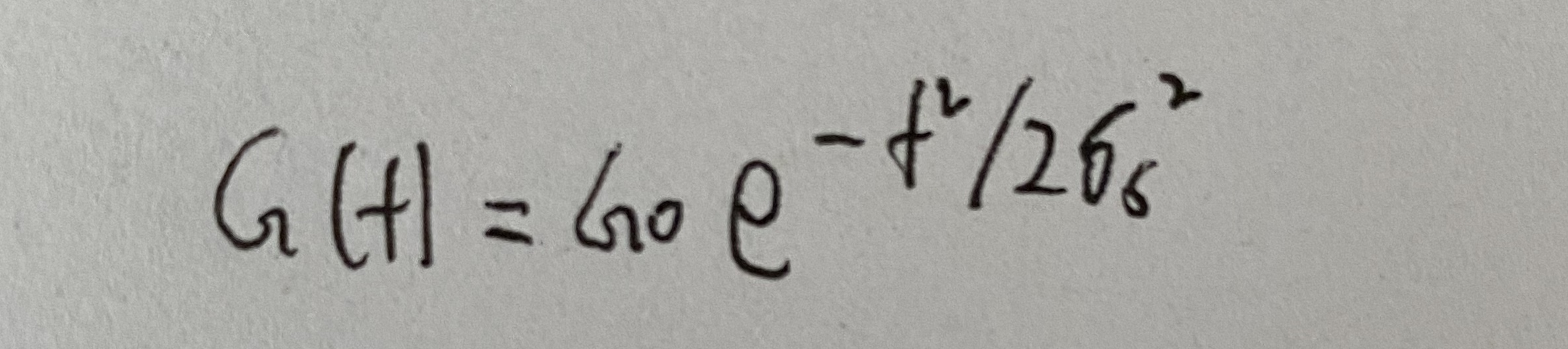2. 动目标显示滤波器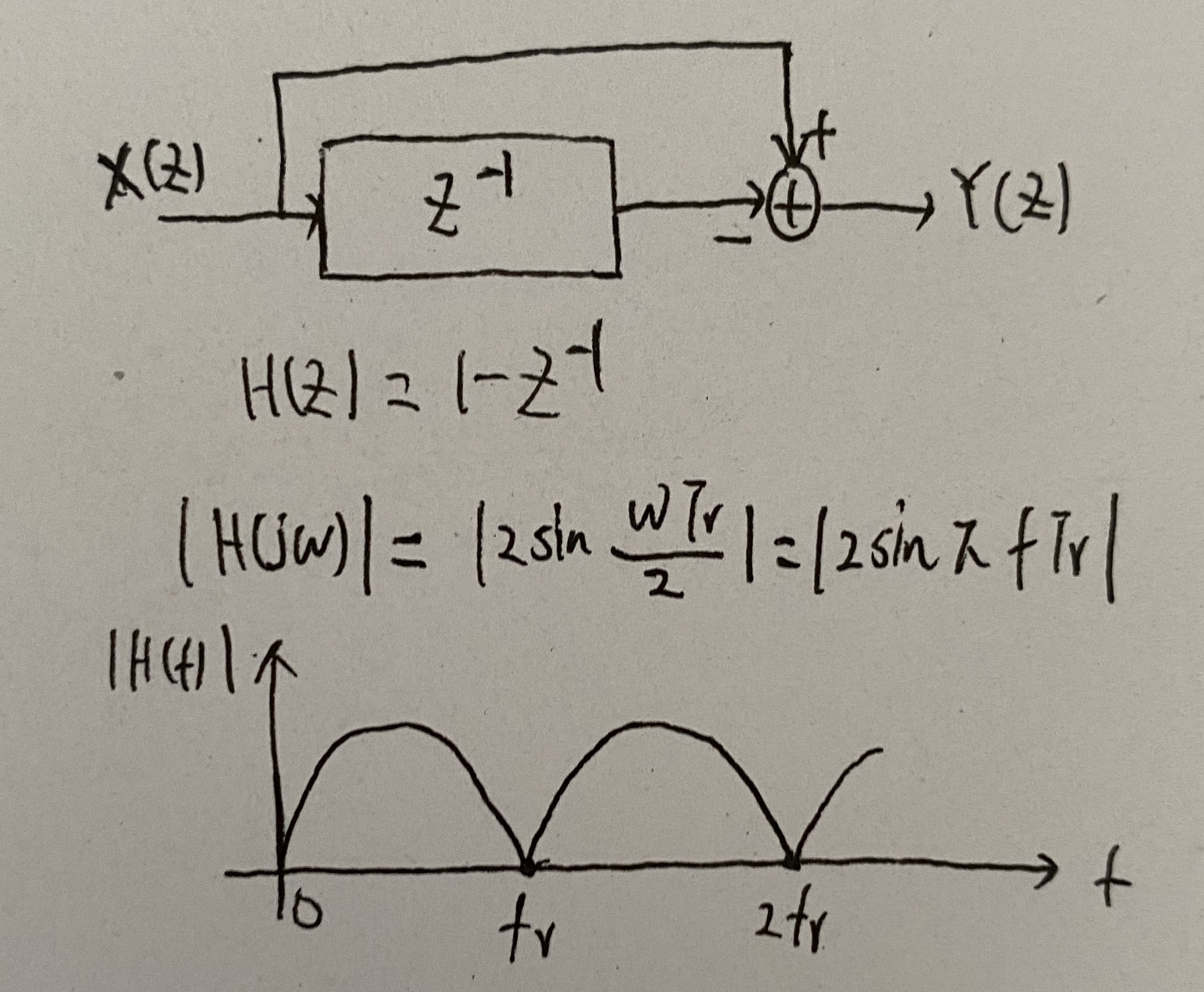动目标检测(MTD)

1. 动目标检测的特点
1. 动态范围更大
2. 改善因子提高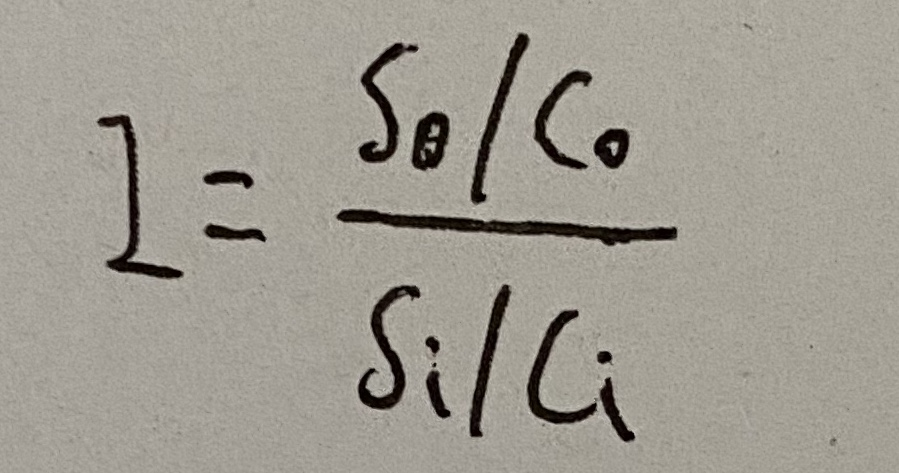3. 增加滤波器组
4. 抑制杂波
5. 增加杂波图
2. 多普勒滤波器组
具有N个输出的横向滤波器(N个脉冲和N-1根延迟线)经过各脉冲不同的加权并求和后，可以做成N个相邻的窄带滤波器组。该滤波器组的频率覆盖范围为0~fr，fr为雷达工作重复频率
如下图所示(横向滤波器)：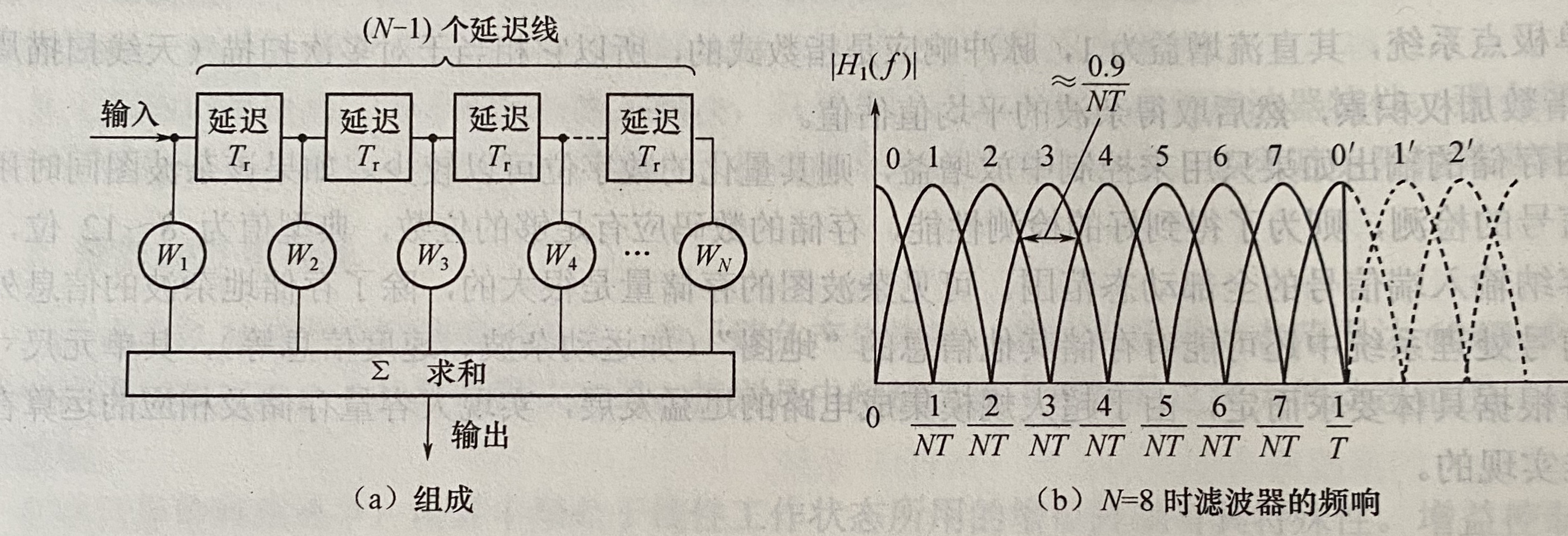横向滤波器有N-1根延迟线，每根延迟线的延迟时间为Tr=1/fr。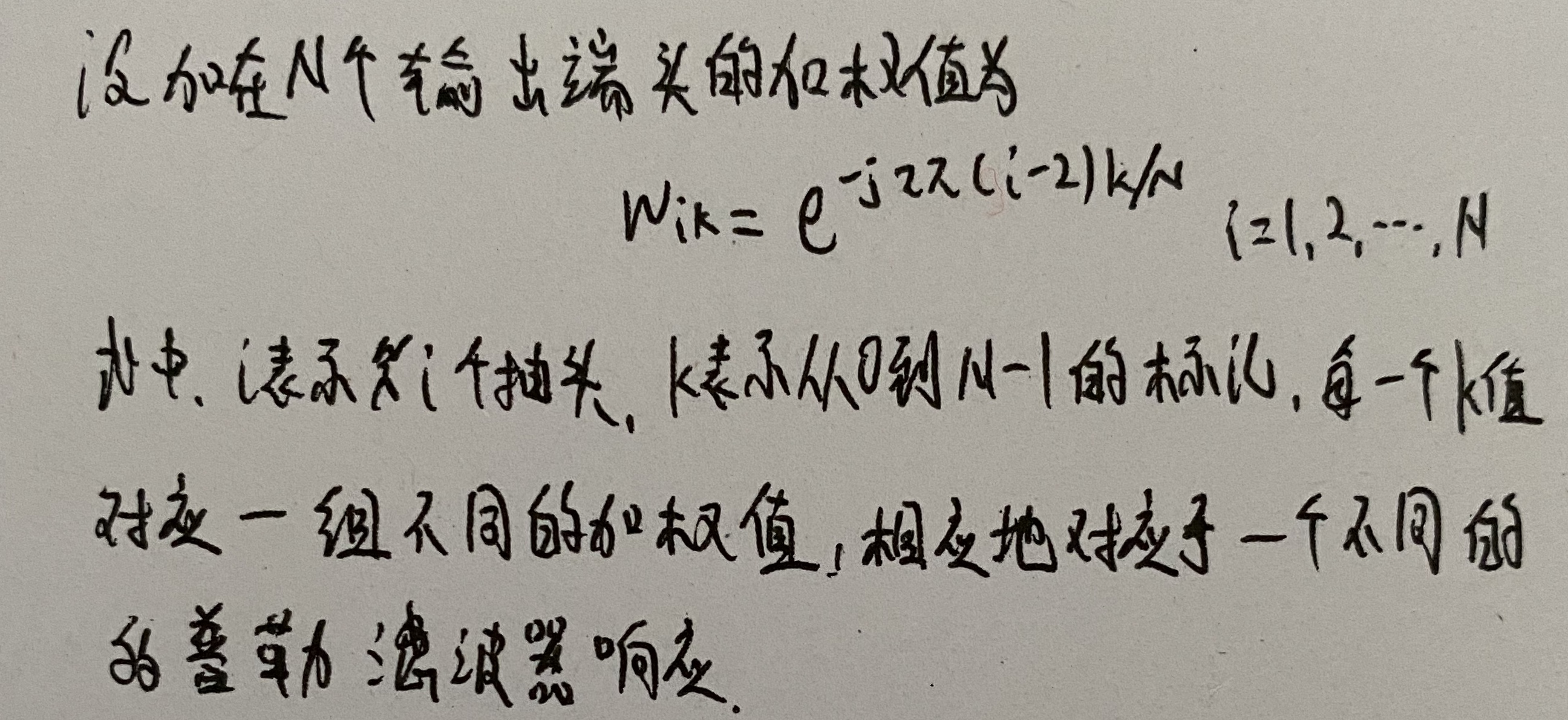上图b表示的是N=8时滤波器所得各标记k的滤波器频率响应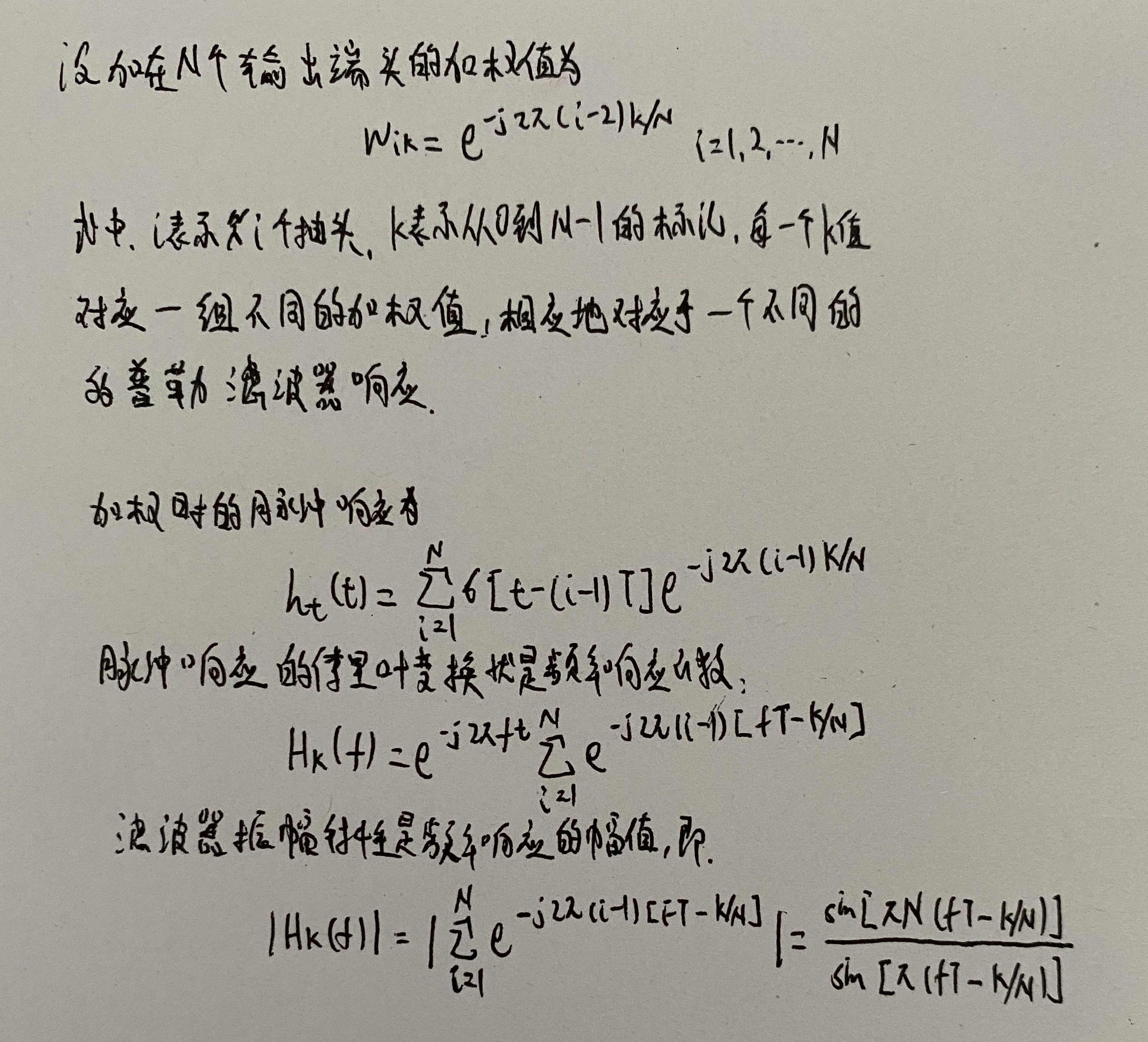当幸福来敲门

下面的是笔者的微信公众号，欢迎关注，会持续更新c++、python、tensorflow、机器学习、深度学习、计算机视觉、雷达原理等系列文章。展开全文• 基于线性调频（LFM）脉冲压缩雷达原理及雷达动目标显示（MTI）的数学模型，通过与传统二脉冲对消和三脉冲对消的方法相对比，采用四脉冲对消和参差脉冲重复频率处理盲速的方法进行动目标检测。仿真实验证明，在动目标...
• 带宽：用来标识信号传输的数据传输能力、标识单位时间内通过链路的数据量、标识显示器的显示能力。 负载：承载的传输数据量。 时延：指一个报文或分组从一个网络的一端传送到另一个端所需要的时间 可靠性：...RIP 动态路由 RIP工作原理 RIP配置
• 动态多目标算法之（基于预测的方法） 其中基于预测的方法中，也有很多在PPS算法的基础上，进行一些改进，最终也可以显示出提高收敛速度的能力。 C、决策变量分类方法 通过结合多样性引入和基于快速预测的方法来利用...算法 机器学习 自然语言处理 经验分享 ieee论文
• 运动目标跟踪（九）--Struck跟踪原理

万次阅读 多人点赞 2016-08-16 14:25:46
《Struck:Structured Output Tracking with Kernels》是 Sam ...Struck与传统跟踪算法的不同之处在于：传统跟踪算法(下图右手边)将跟踪问题转化为一个分类问题，并通过在线学习技术更新目标模型。然而
• 对于RD算法的原理这里就不展开讨论了，重点分析距离徙校正的原理。 仿真程序，F_s=2.5B，距离徙最大2.92m，对应7.3个距离单元。PRF取1.2倍多普勒带宽，目标点相对于参考点的位置为(-50,50,0)，则仿真结果如下...雷达成像 RD算法 距离徙动校正 matlab
• 简单来说就是一秒钟，屏幕显示多少张画面。 为什么是 60fps ？ 人类视觉的时间敏感度和分辨率根据视觉刺激的类型和特征而变化，并且在个体之间不同。人类视觉系统每秒可处理10到12个图像并单独感知它们，而较高的...android 渲染 原理
• 动态视频目标检测和跟踪技术 http://m.qingqingsk.com/ztnews/lvvozlzrztkzrqwqqlnrluqk.html 传统电视监控技术只能达到“千里眼”的作用，把远程的目标图像（原始数据）传送到监控中心，由监控人员根据目视...
• OLED显示模块驱动原理及应用

万次阅读 多人点赞 2018-12-10 10:15:03
OLED显示模块驱动原理及应用   本文以中景园OLED显示模块为例，介绍模块的应用和OLED显示及驱动的基本原理。文中介绍了显示模块、SSD1306驱动芯片以及GT20L16S1Y字库芯片相关技术内容及原理，并加上了作者的理解...
• opencv动态目标跟踪学习总结

万次阅读 多人点赞 2016-03-15 11:03:49
用opencv实现对视频中动态目标的追踪 第一步，是要建立一个编程环境，然后加载opencv的库路径等等。具体步骤在 http://www.opencv.org.cn/ 的“安装”中 有详细介绍。 第二步，建立一个MFC的对话框程序，做两个...opencv 视频目标跟踪
• DWA动态窗口法的原理及应用

千次阅读 多人点赞 2020-04-03 20:56:22
对于cost function, 我先前就写过一个更详细的： 自动驾驶路径规划DWA算法原理解析 这个里面直接列出了7项cost function: 这里，我们介绍的时候还是按照最简单的来做，代码实现也仅包含上述的三项基础部分。...
• Java 8支持动态语言，看到了很酷的Lambda表达式，对一直以静态类型语言自居的Java，让人看到了Java虚拟机可以支持动态语言的目标java 动态语言 Lambda表达式
• 主要介绍当前主要目标运动检测方法的原理和利用，对这些算法有一个初步了解，文章中设计借鉴其他文章，在这里一一感谢。算法
• 在前一文中，我们分析了Activity组件的切换过程。从这个过程可以知道，所有参与切换操作的窗口都会被设置切换动画。事实上，一个窗口在打开（关闭）的...本文就详细分析WindowManagerService服务显示窗口动画的原理Android Activity Animation 动画
• TLD目标跟踪原理解析

千次阅读 2016-09-21 19:16:12
TLD(Tracking-Learning-Detection)是英国萨里大学的一个捷克籍博士生在其攻读博士学位期间提出的一种新的单目标长时间（long term tracking）跟踪算法。该算法与传统跟踪算法的显著区别在于将传统的跟踪算法和传统...
• 【目标追踪】python帧差法原理及其实现

千次阅读 多人点赞 2020-01-05 21:55:52
python基于帧差法的视频目标追踪帧差法目标追踪原理1. 基本思路：2. 图例讲解：3. 存在的问题&如何解决：第一步——调用摄像头：1. 使用opencv打开摄像头：2. 读取逐帧图片：第二步——处理图片：1. 转换成灰度...opencv 计算机视觉 图像识别 深度学习 目标检测
• 基于自适应颜色属性的目标追踪 Adaptive Color Attributes for Real-Time Visual Tracking 基于自适应颜色属性的实时视觉追踪  3月讲的第一篇论文，个人理解，存在很多问题，欢迎交流！ 这是CVPR2014
• 目标运动方向判断

千次阅读 2016-06-23 16:36:53
一、原理  在有些场合由于需要检测目标的运动方向，判断是进入检测区域或是离开检测区域，因此需要设定警戒线。运动方向的判断一般通过检测目标的运动方向来判断。也就是运用运动估计的思想。运动方向坚持需要用...
• 其基本原理就是采用不同大小和比例（宽高比）的窗口在整张图片上以一定的步长进行滑动，然后对这些窗口对应的区域做图像分类，这样就可以实现对整张图片的检测了，如下图3所示，如DPM就是采用这种思路。但是这个方法...目标检测Yolo
• 雷达原理》分为雷达主要分机及测量方法两...运动目标检测部分对强杂波中提取运动目标信号的基本工作原理、精巧的信号处理技术及实现方法均有较深入的讨论，并涉及动目标显示（MTI）及脉冲多普勒（PD）体制的基本原理雷达原理
• 机器视觉 OpenCV—python目标跟踪（光流）

万次阅读 多人点赞 2018-12-05 16:59:11
目标跟踪是对摄像头视频中的移动目标进行定位的过程。实时目标跟踪是许多计算机视觉应用的重要任务，如监控、基于感知的用户界面、增强现实、基于对象的视频压缩以及辅助驾驶等。 好久之前做过一次人脸检测，里面...
• 的主要原理就是用一个随机感知矩阵去降维一个高维信号，得到的低维信号可以完全保持高维信号的特性。这个随机感知矩阵要满足 CS 理论的 RIP 条件就可以完全从低维信号重建高维信号。   二、主要思想：  ...
• 运动目标检测部分对强杂波中提取运动目标信号的基本工作原理、精巧的信号处理技术及实现方法均有较深入的讨论，并涉及动目标显示(MTI)及脉冲多普勒(PD)体制的基本原理。高分辨力雷达部分讨论了雷达分辨理论、高距离...
• 文章目录一、 基本过程和思想二 、视频理解还有哪些优秀框架三、效果体验~使用手势：python run_gesture_recognition.py健身_跟踪器：卡路里计算三、训练自己数据集...用单帧目标检测做的话，前后语义相关性很差（也有人工智能 深度学习 神经网络 爬虫...